# RD Sharma Solutions for Class 6 Maths Chapter 5: Negative Numbers and Integers

RD Sharma Solutions are considered to be useful for the students of Class 6 to boost their exam preparation. Understanding the basic concepts in Class 6 is vital as it helps us to solve problems in higher grades. Here, we mainly provide detailed exercise wise solutions to help students to solve the problems easily.

The problems which are solved contain explanations in a simple language which help students gain better knowledge about concepts. The solutions are accurate according to the RD Sharma textbook of current CBSE board. RD Sharma Solutions for Class 6 Maths Chapter 5 Negative Numbers and Integers are provided here.

## RD Sharma Solutions for Class 6 Maths Chapter 5: Negative Numbers and Integers Download PDF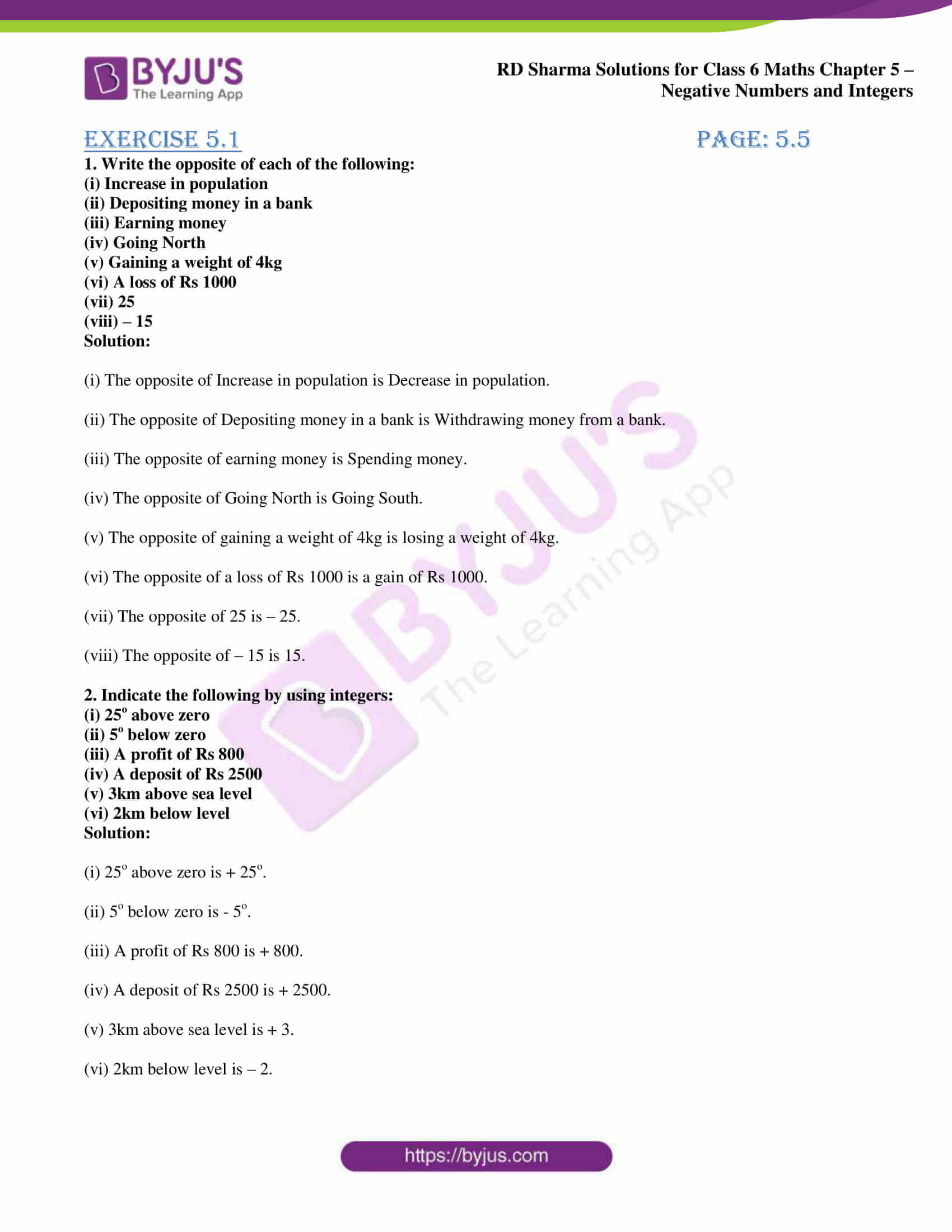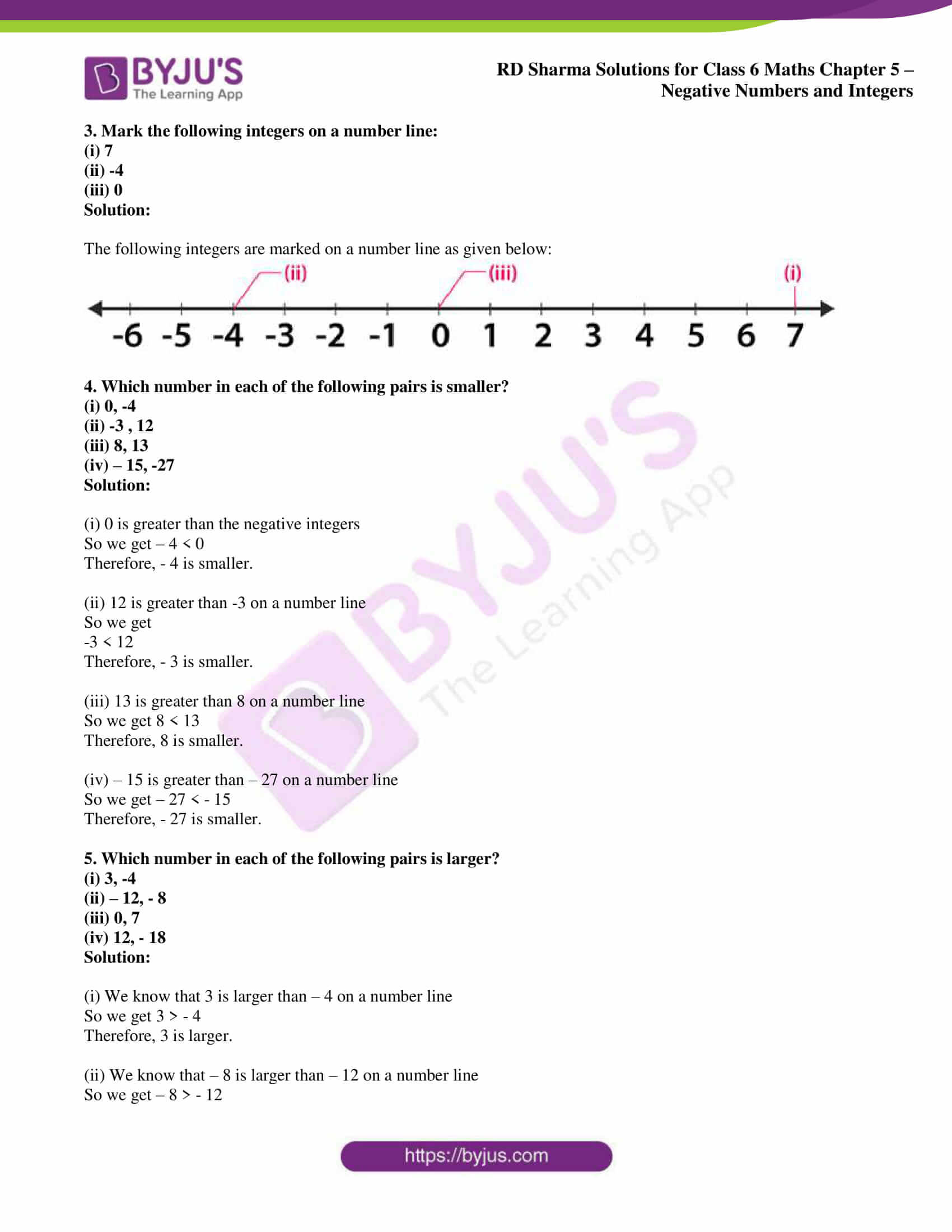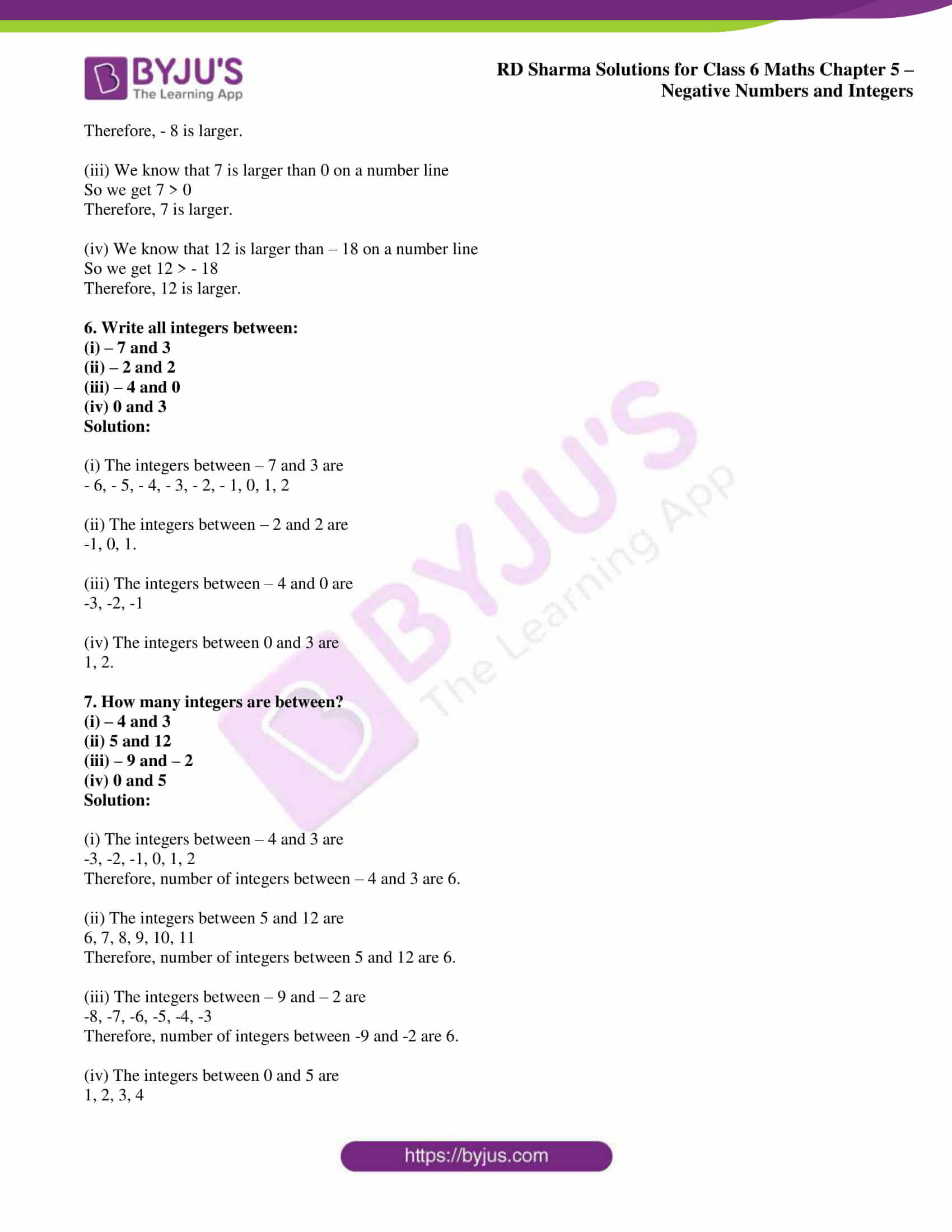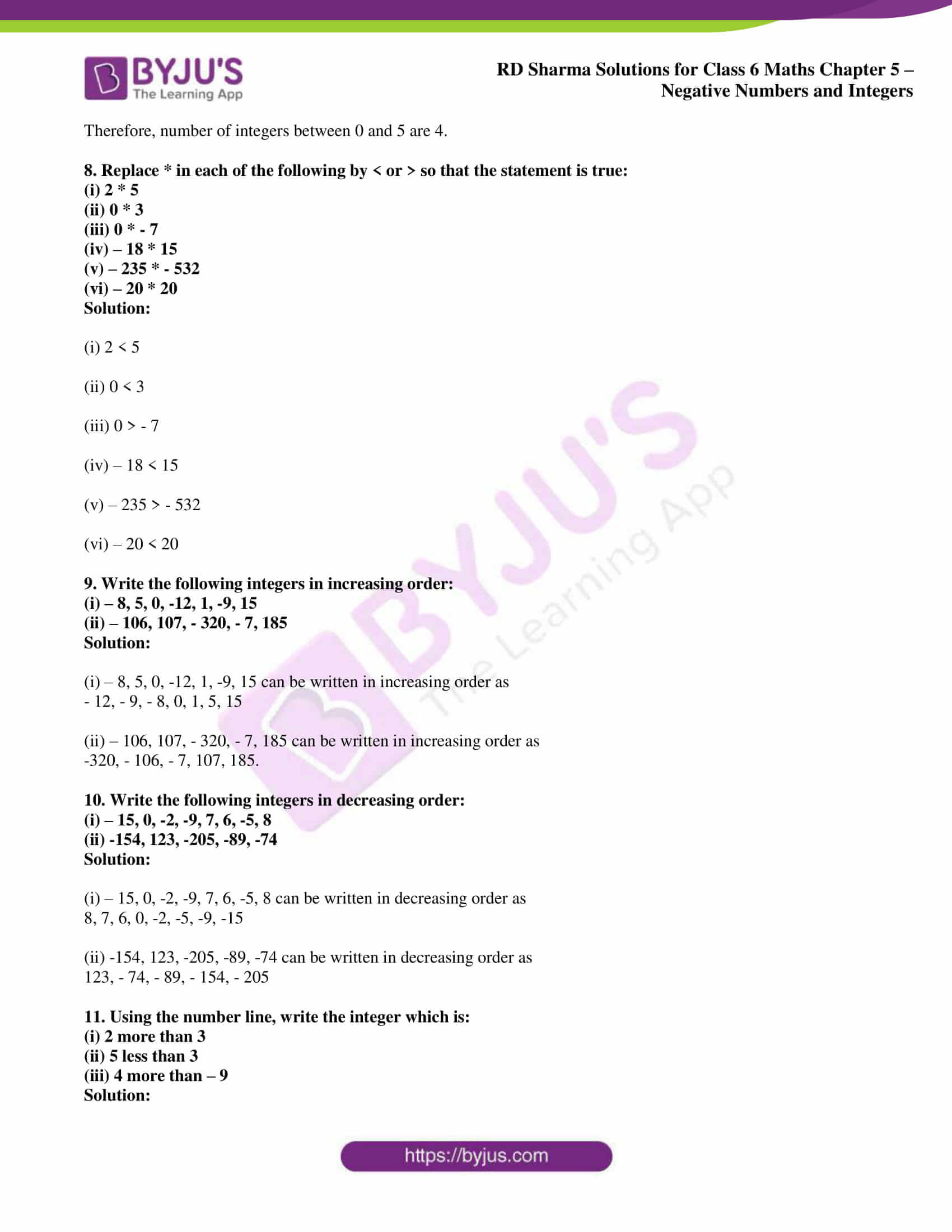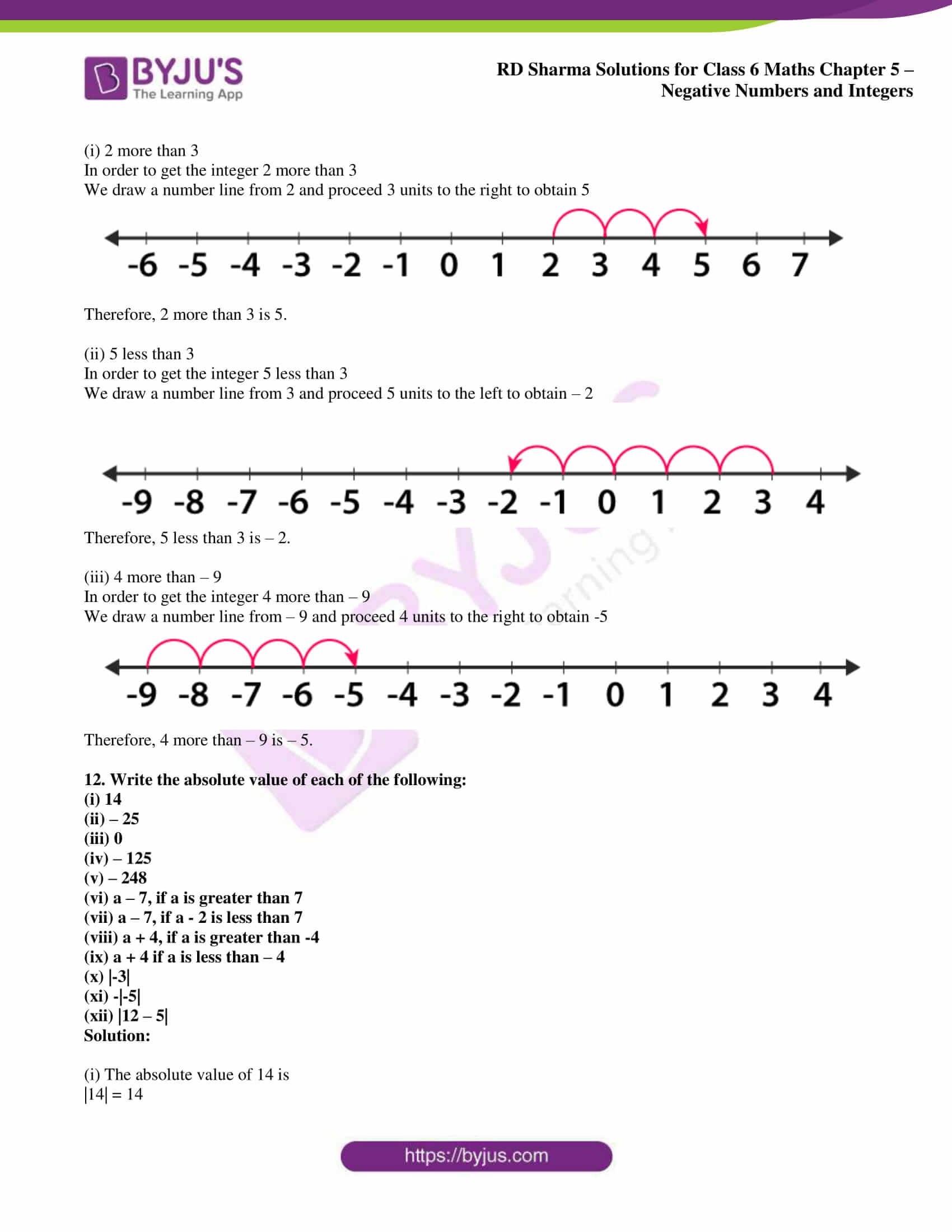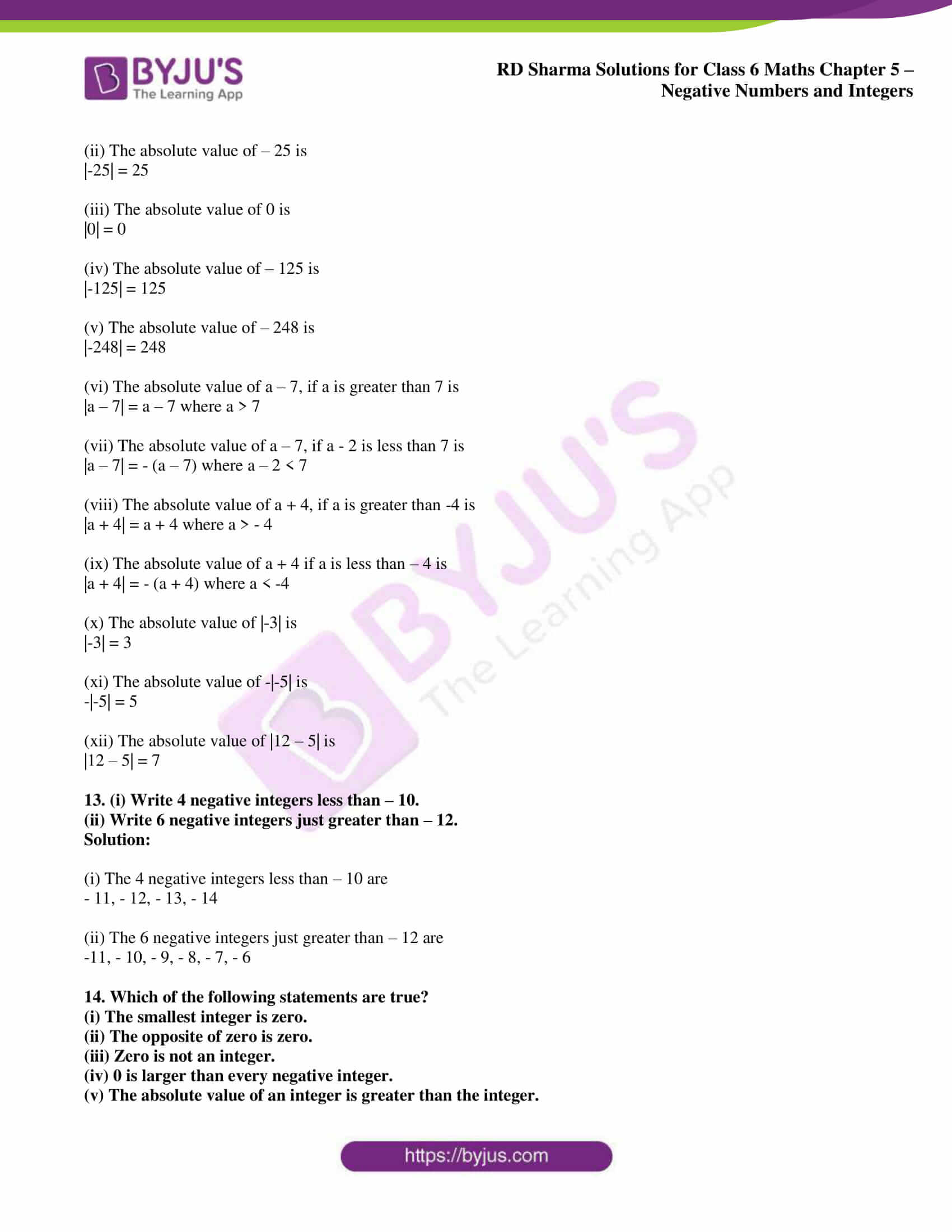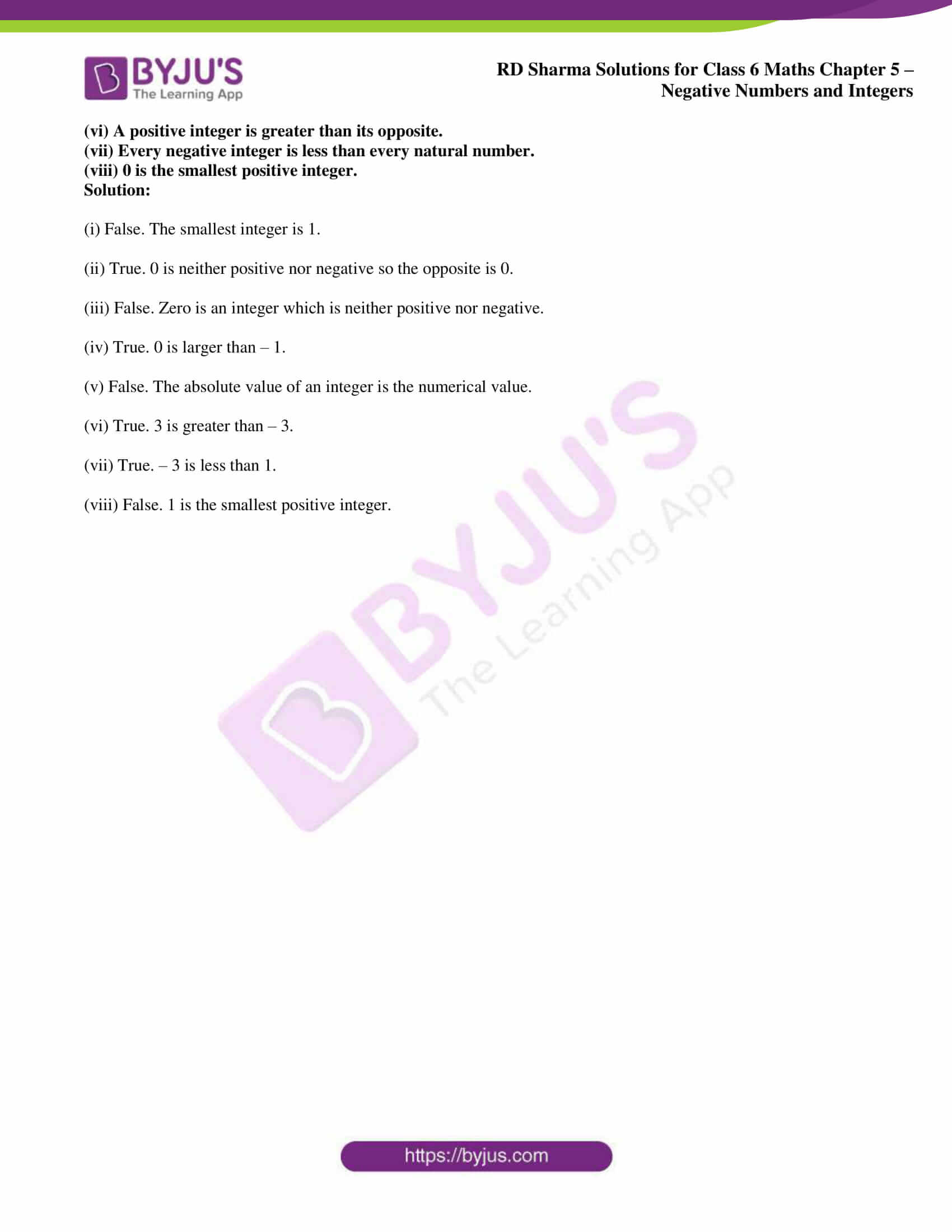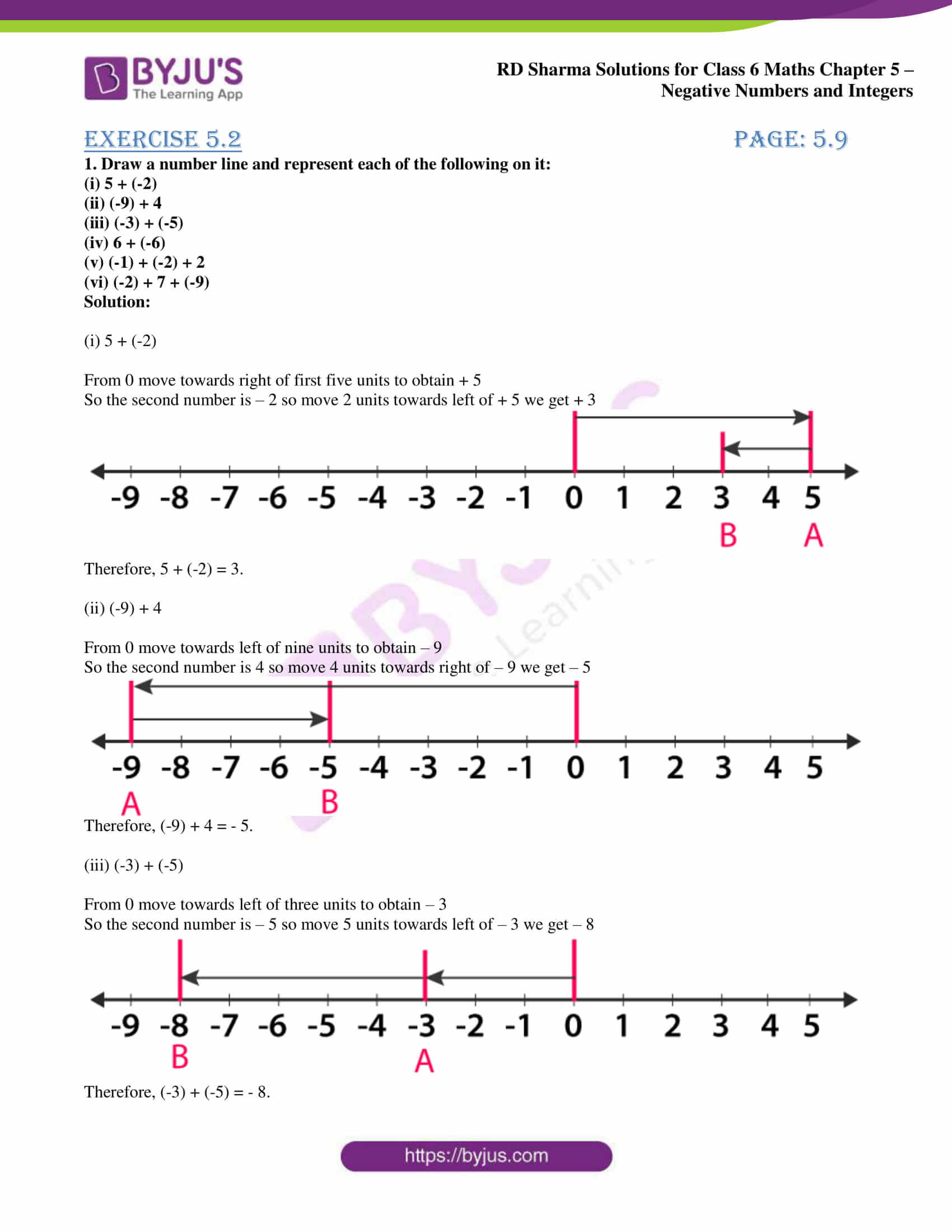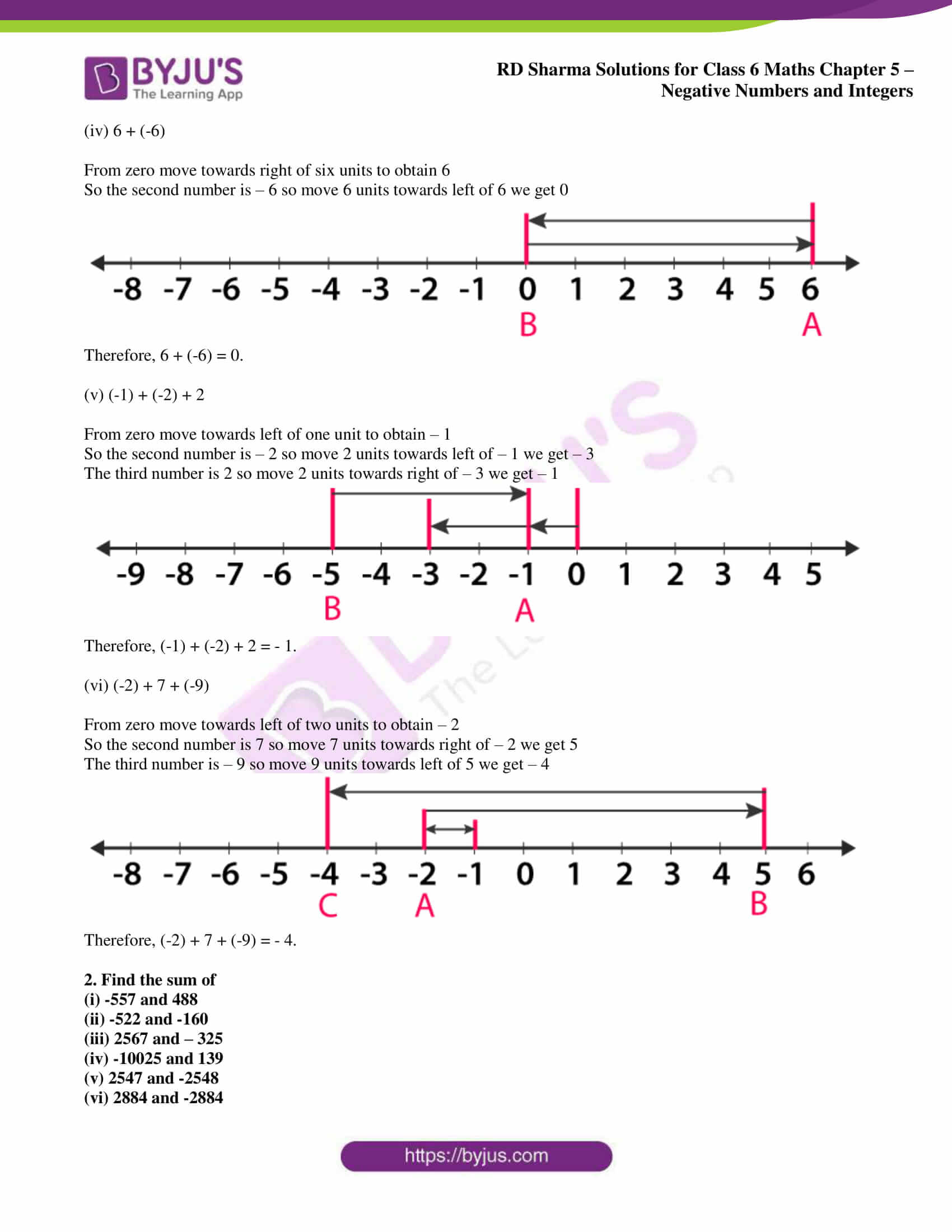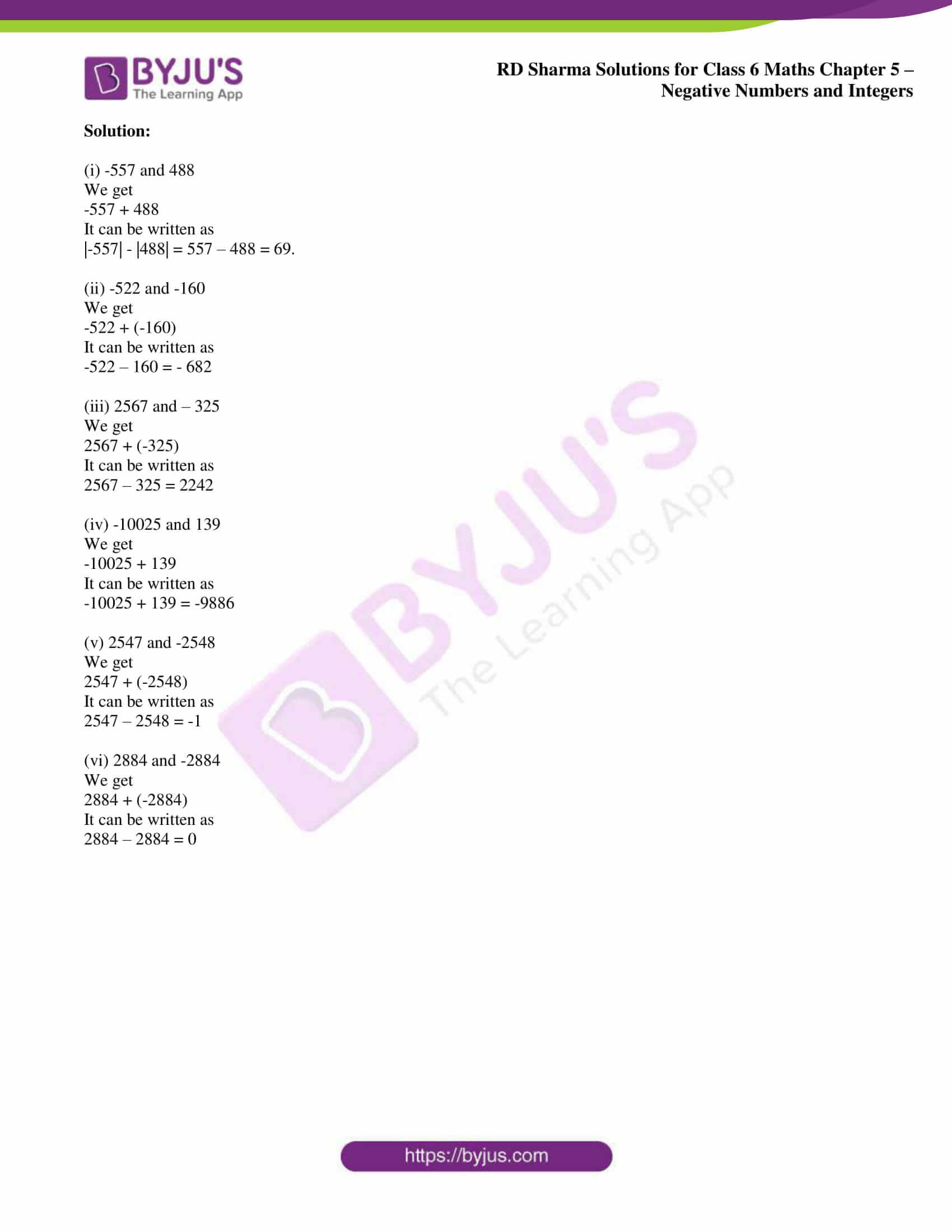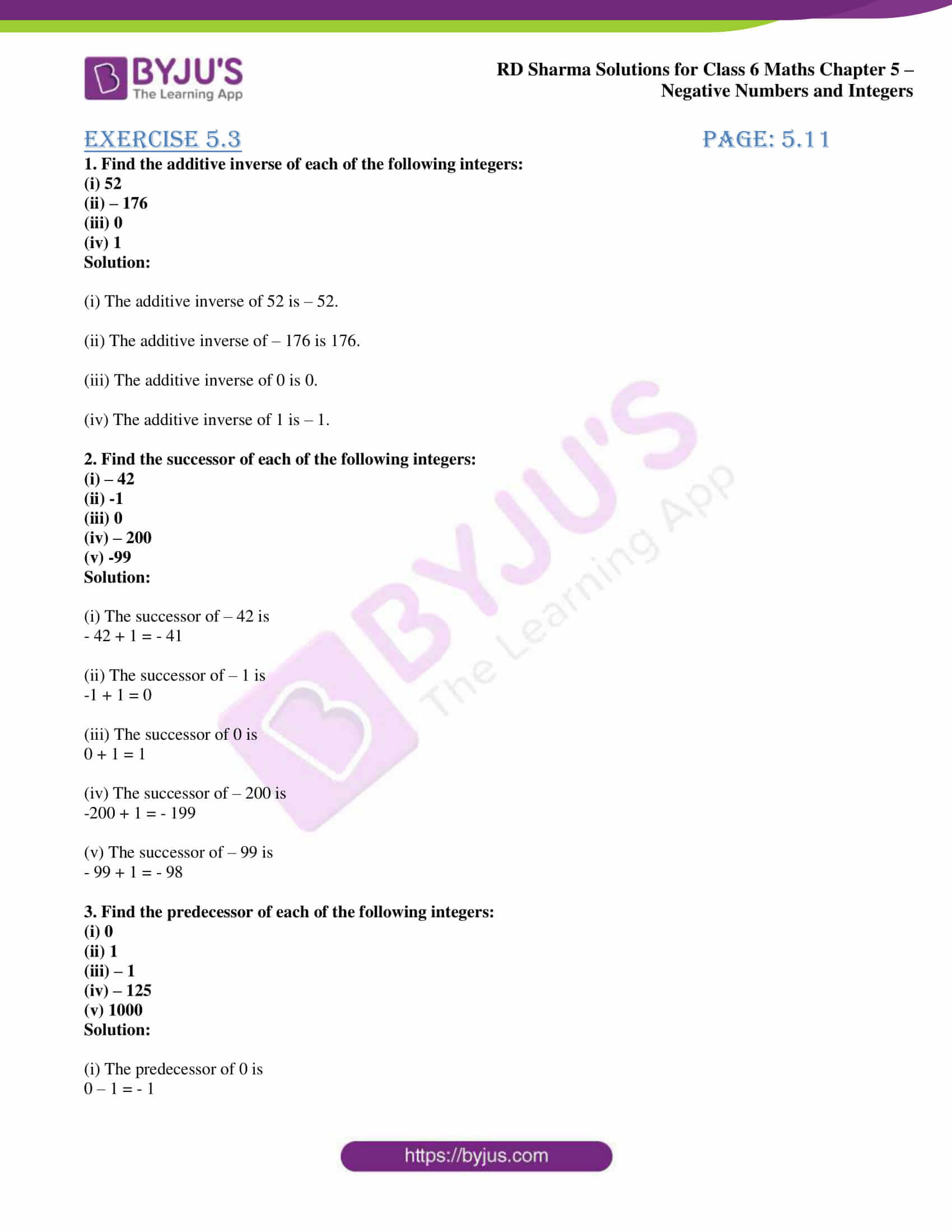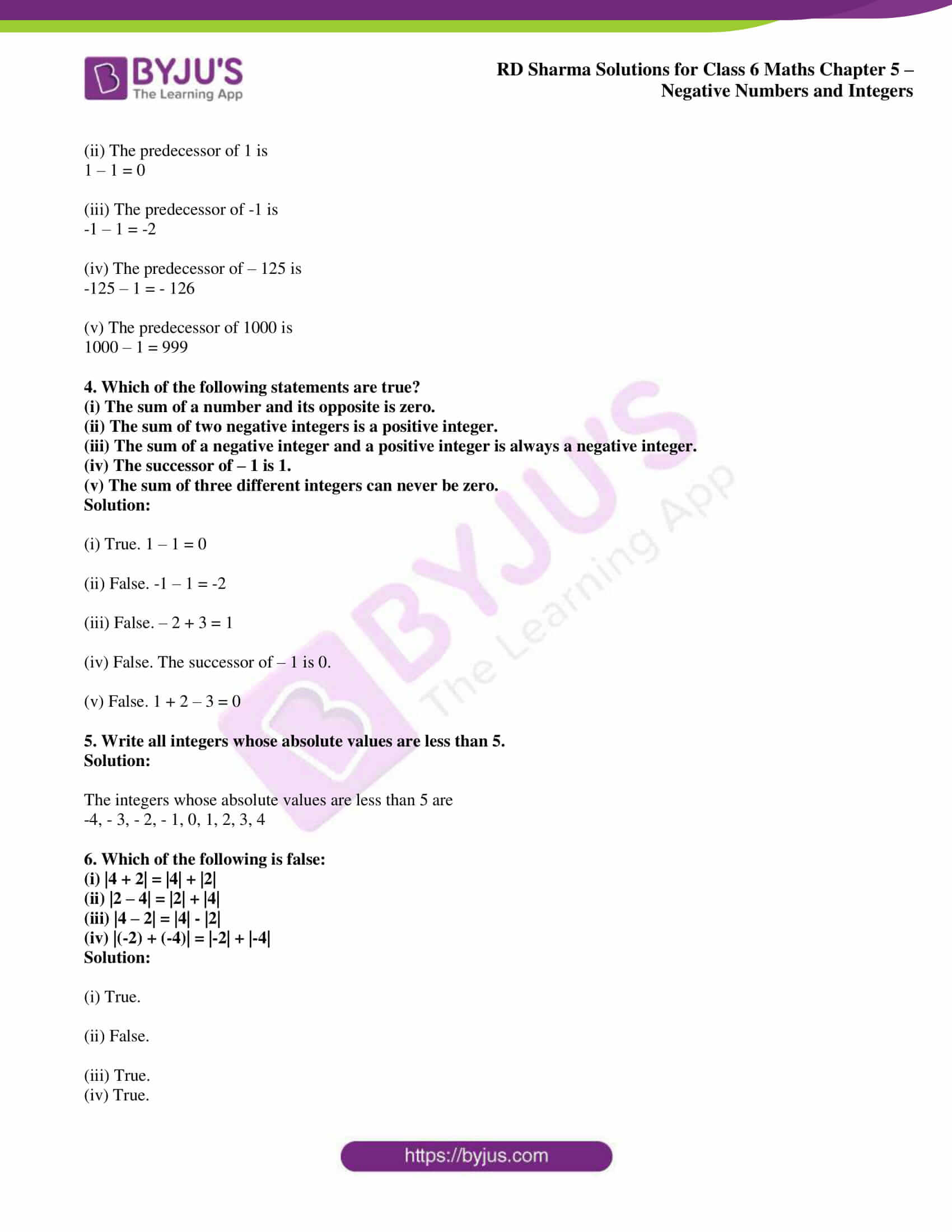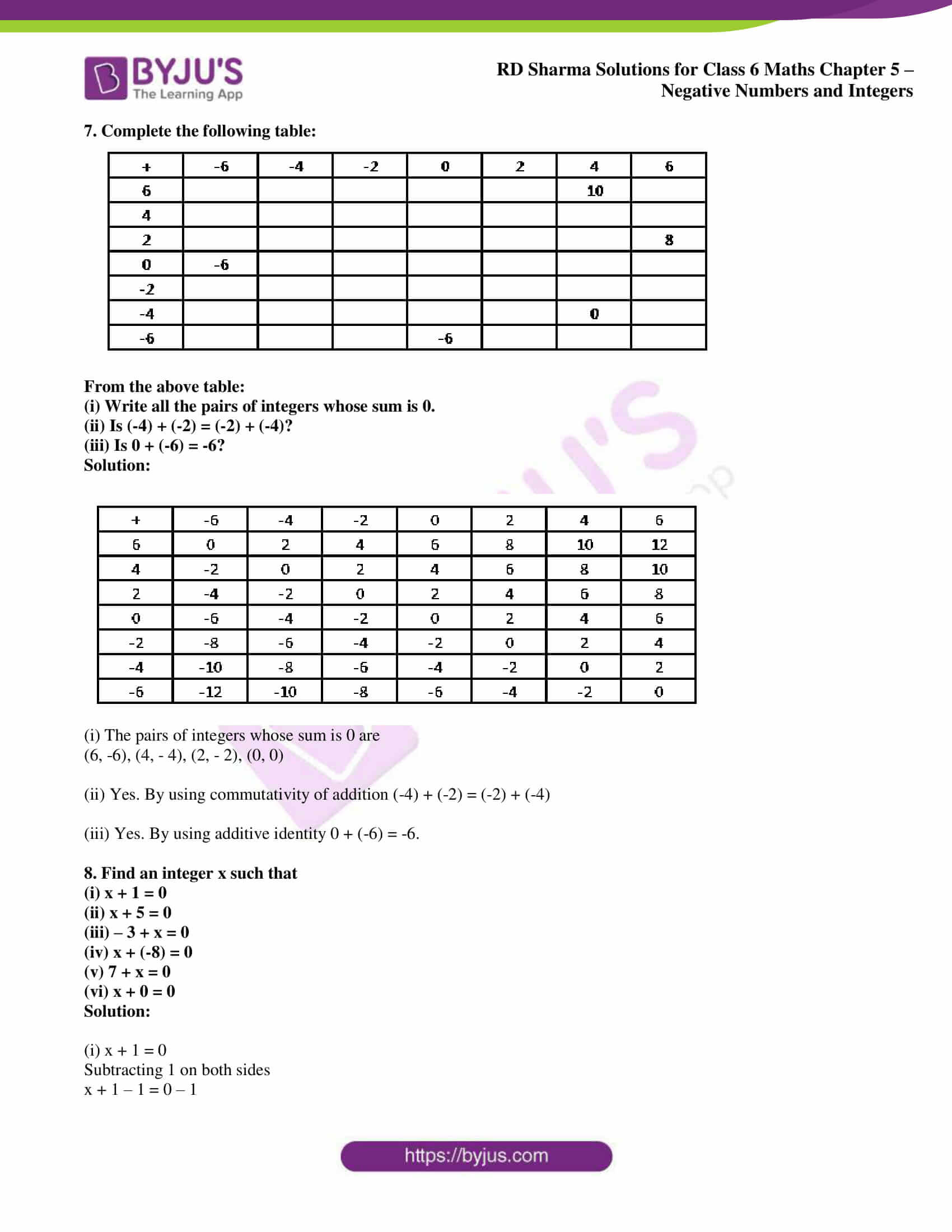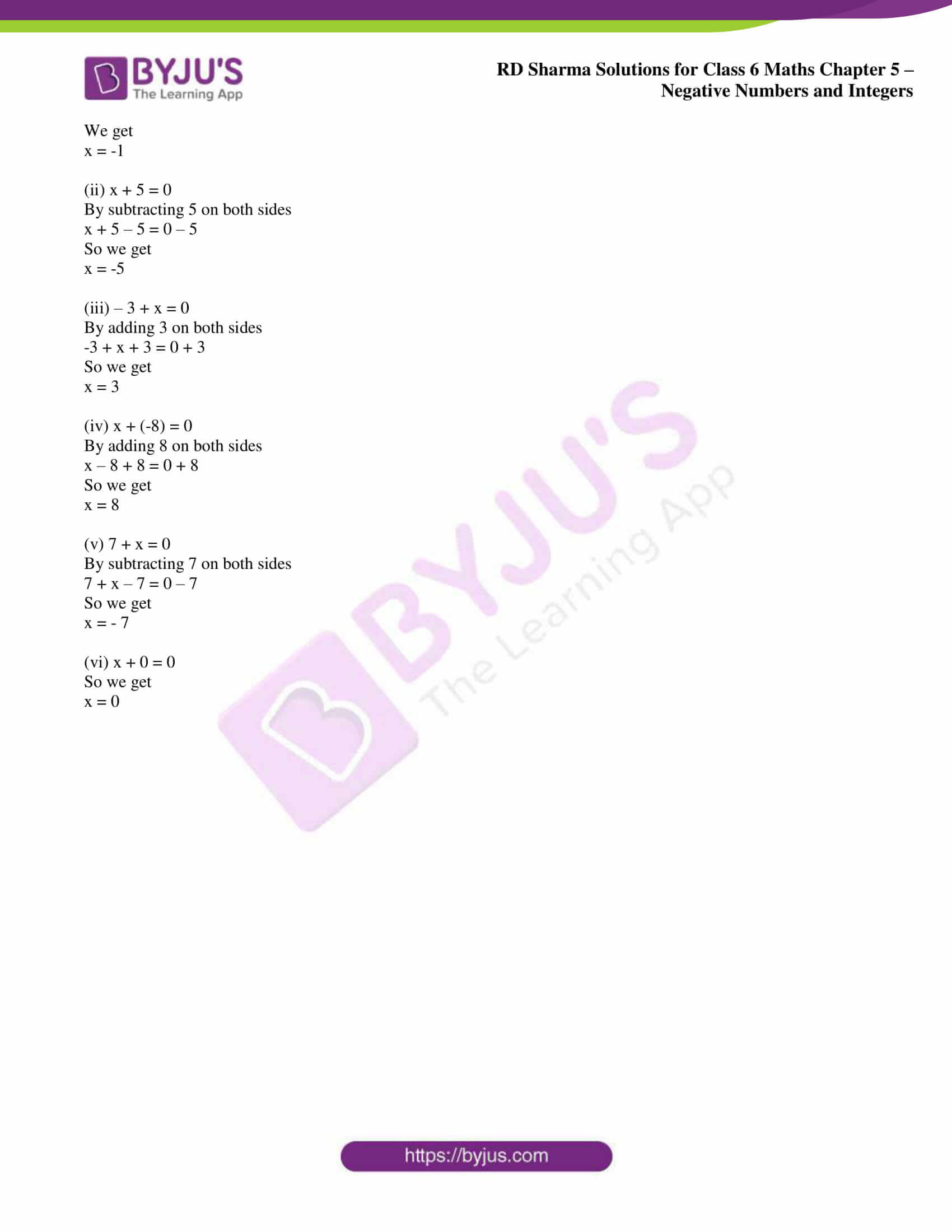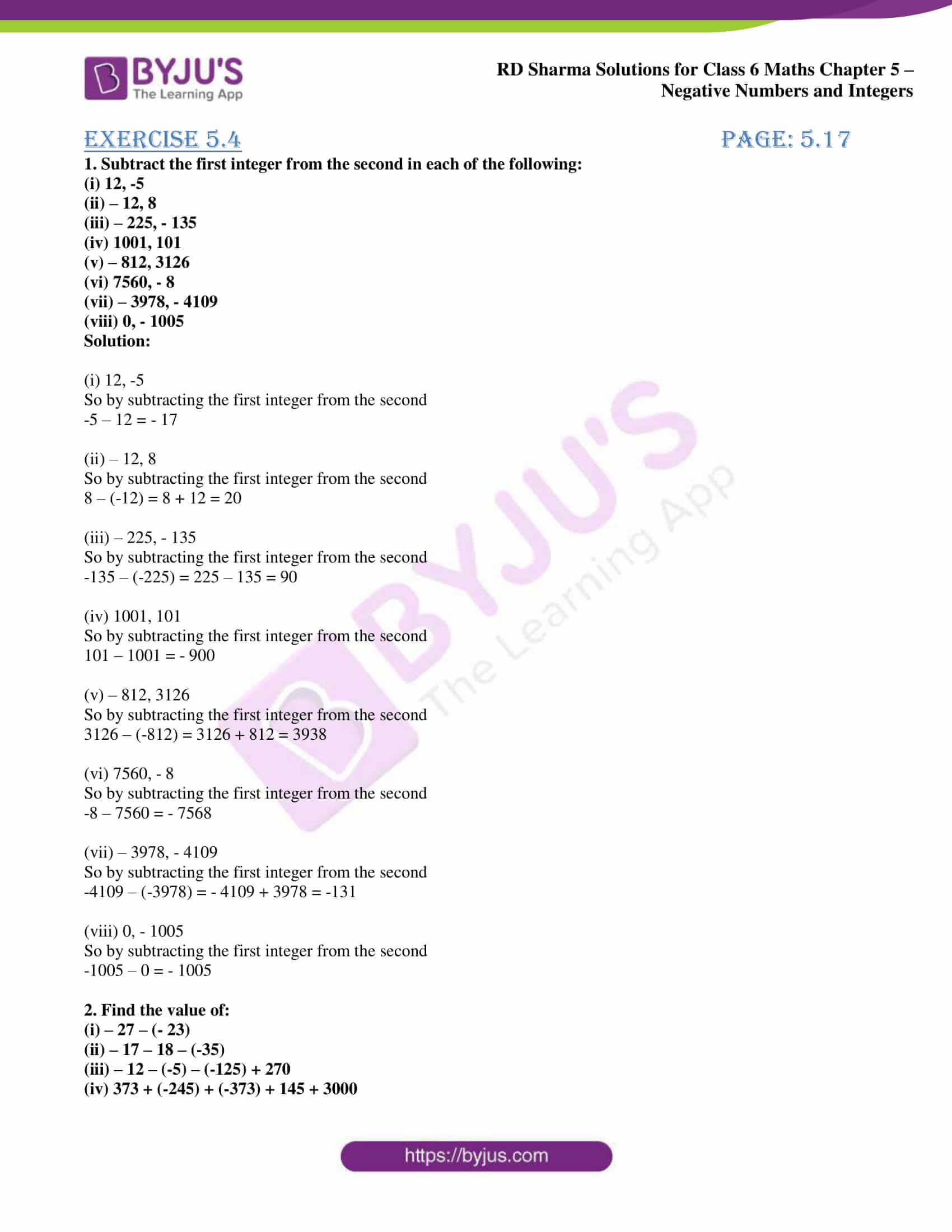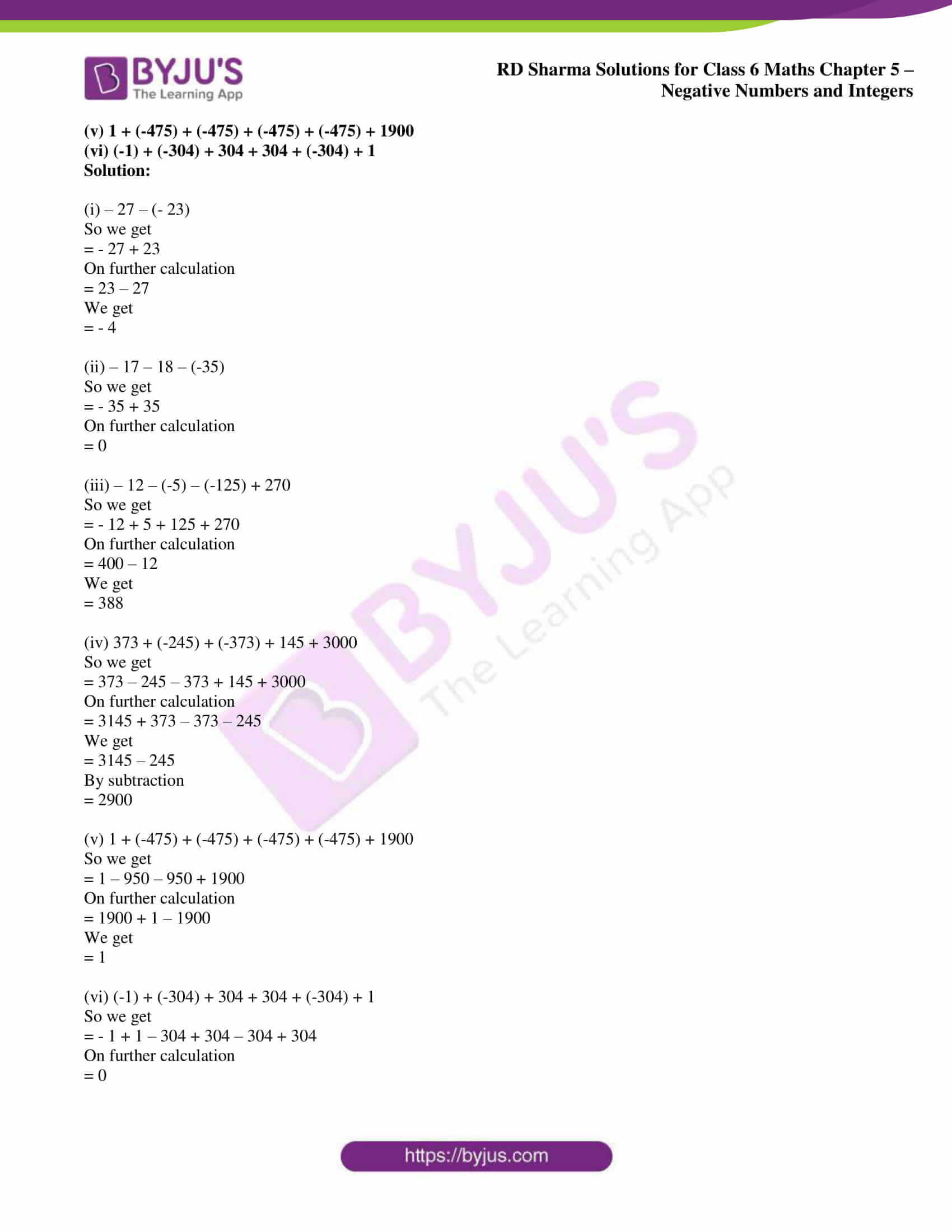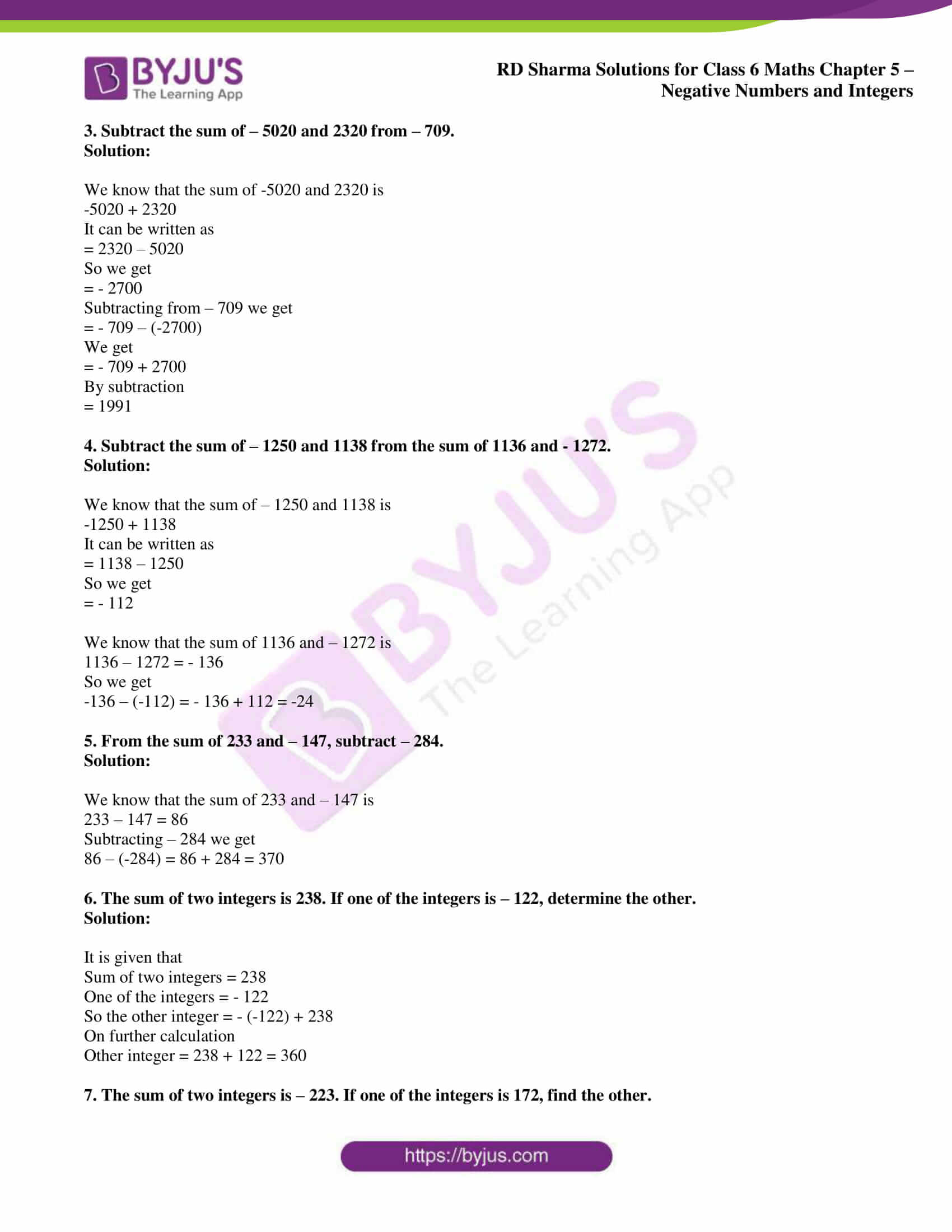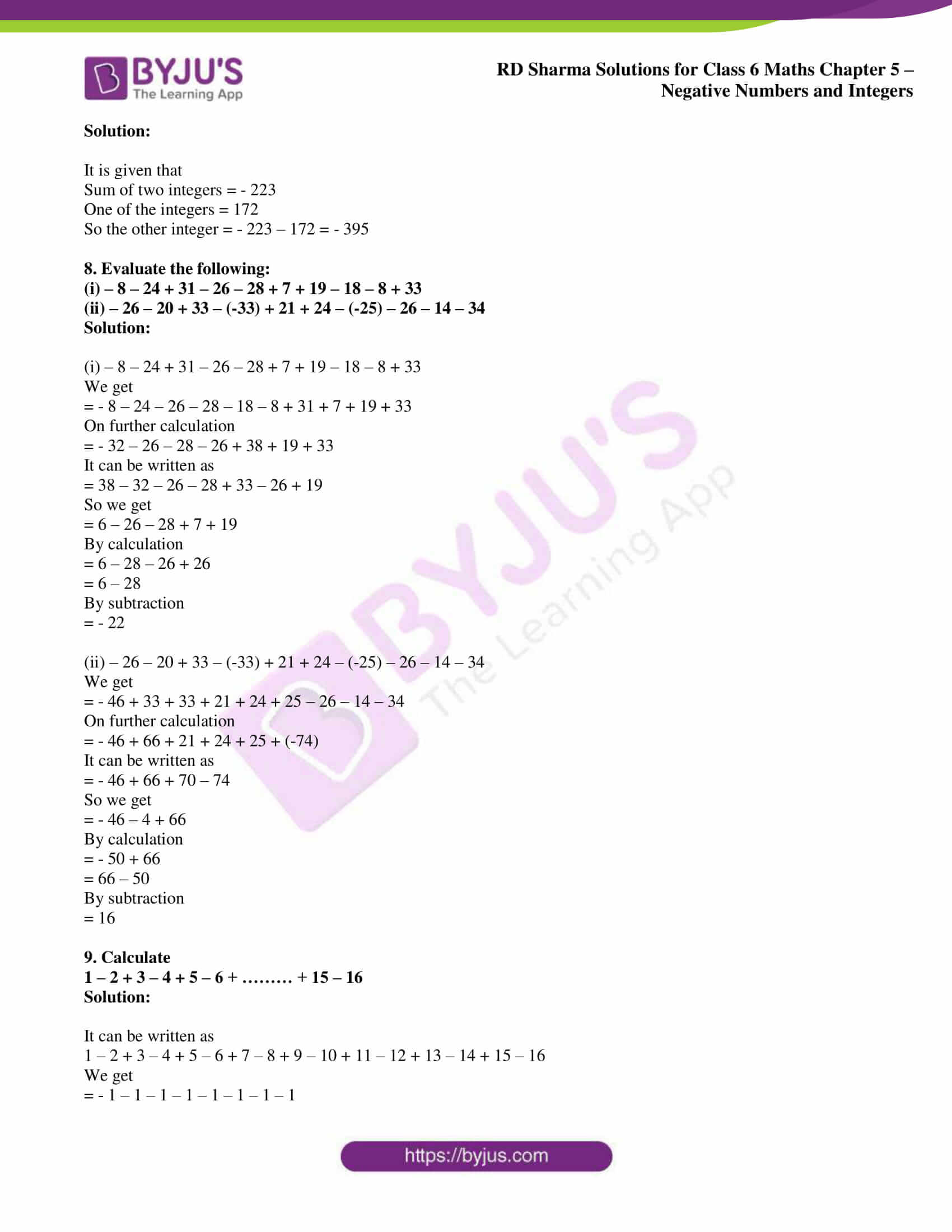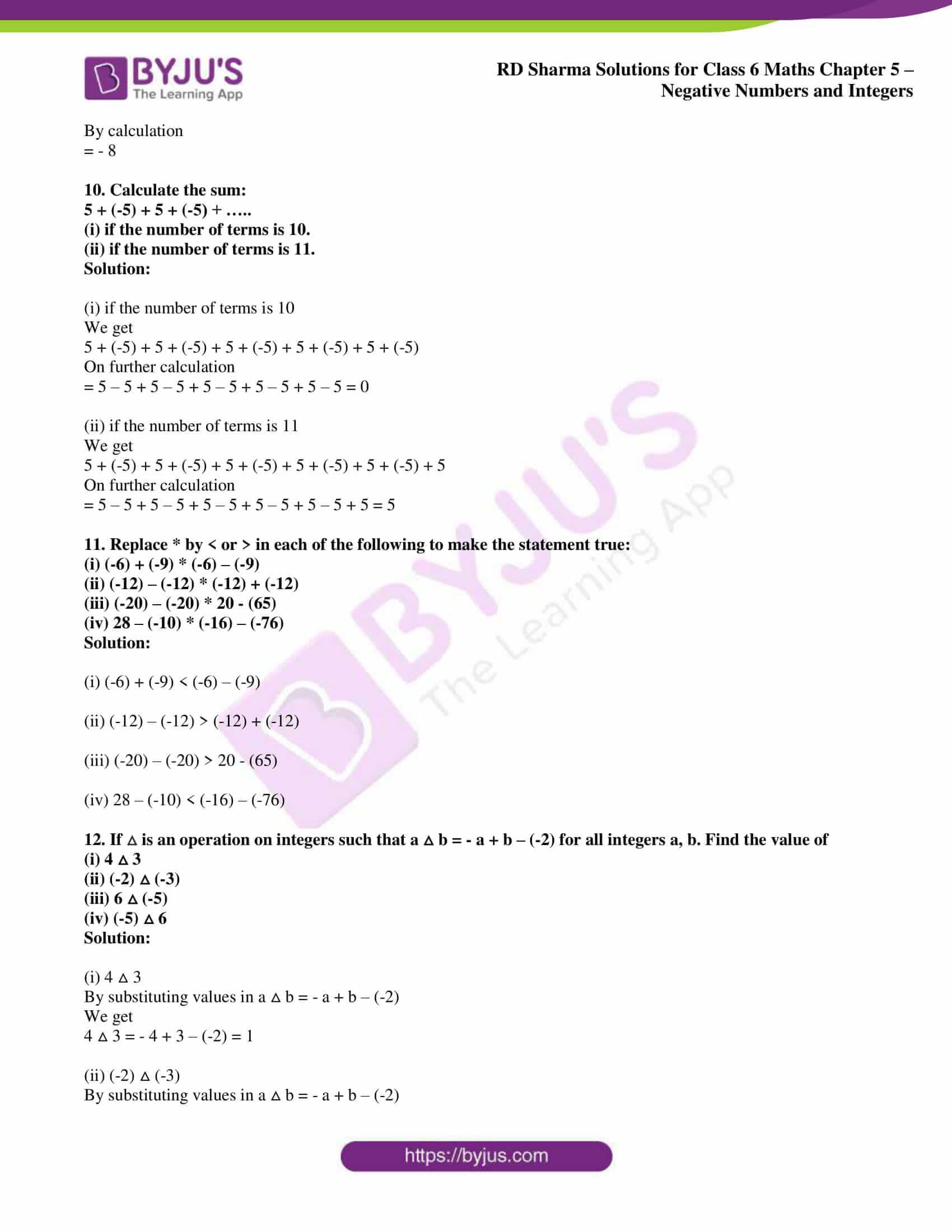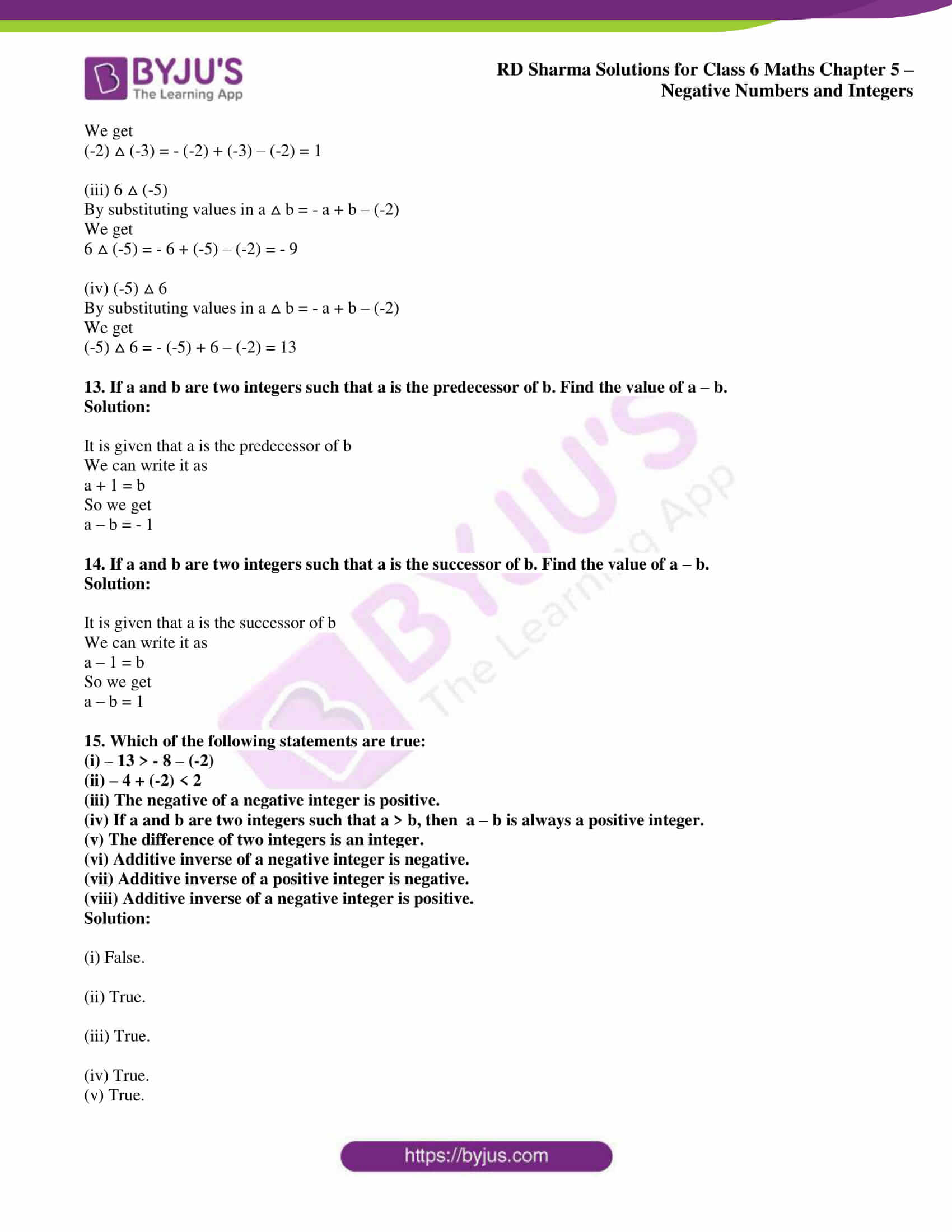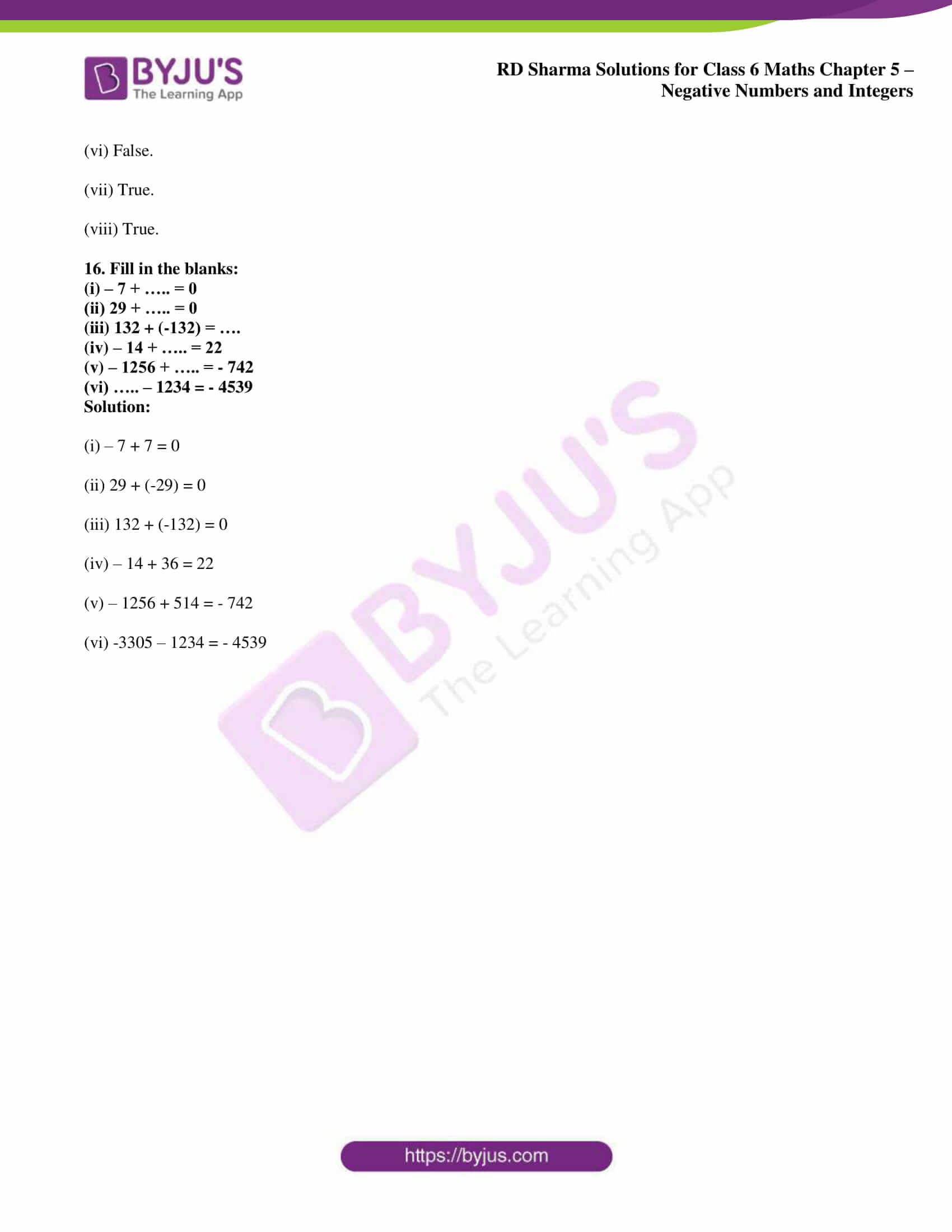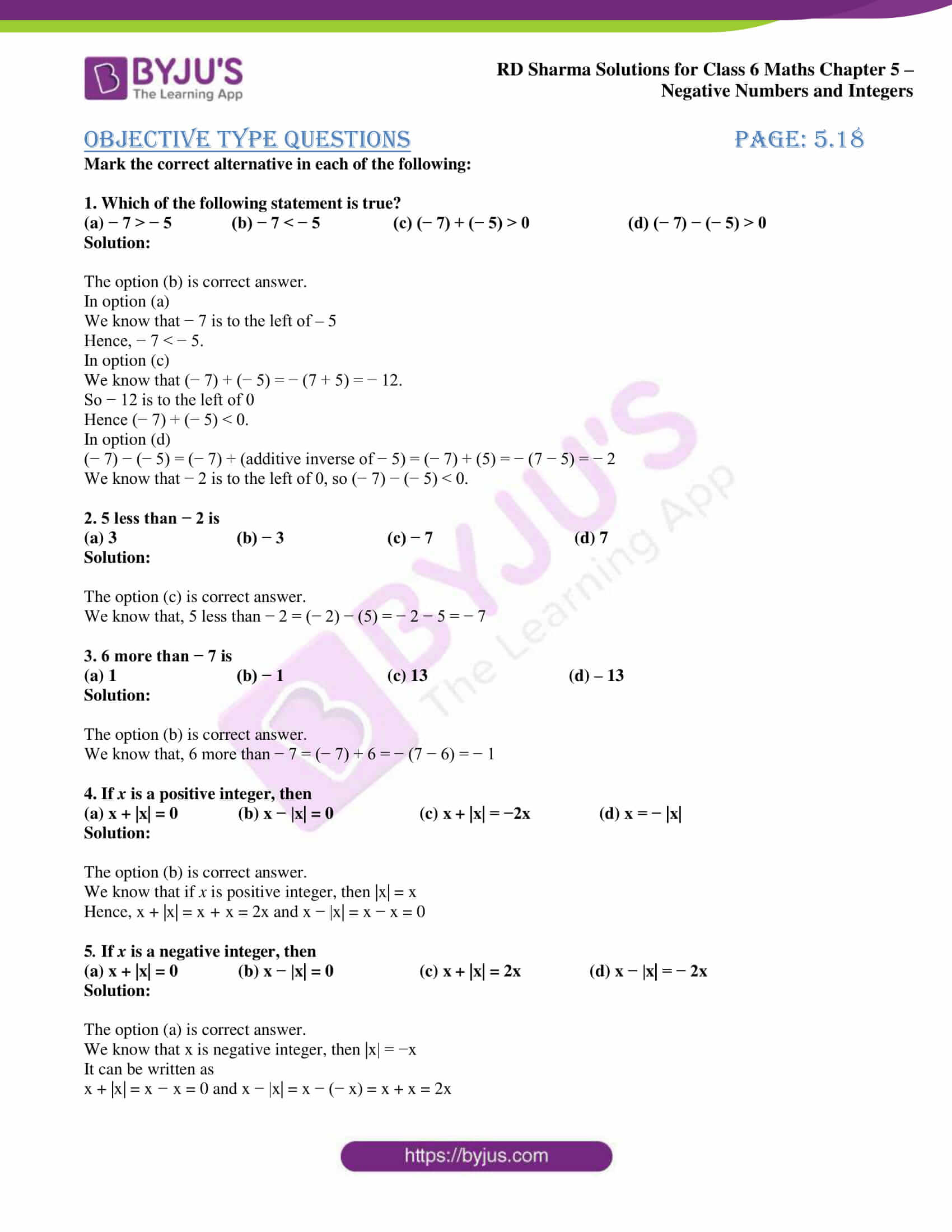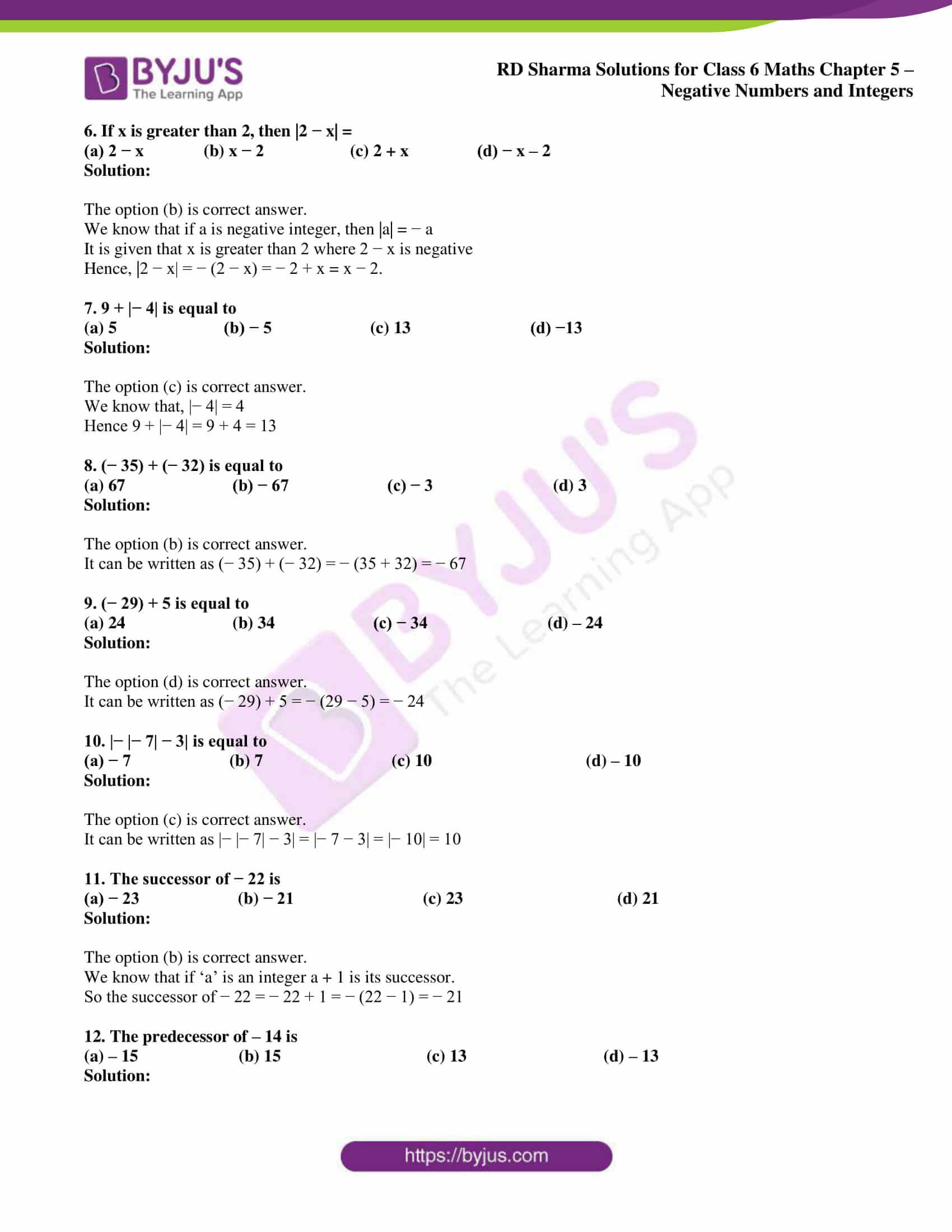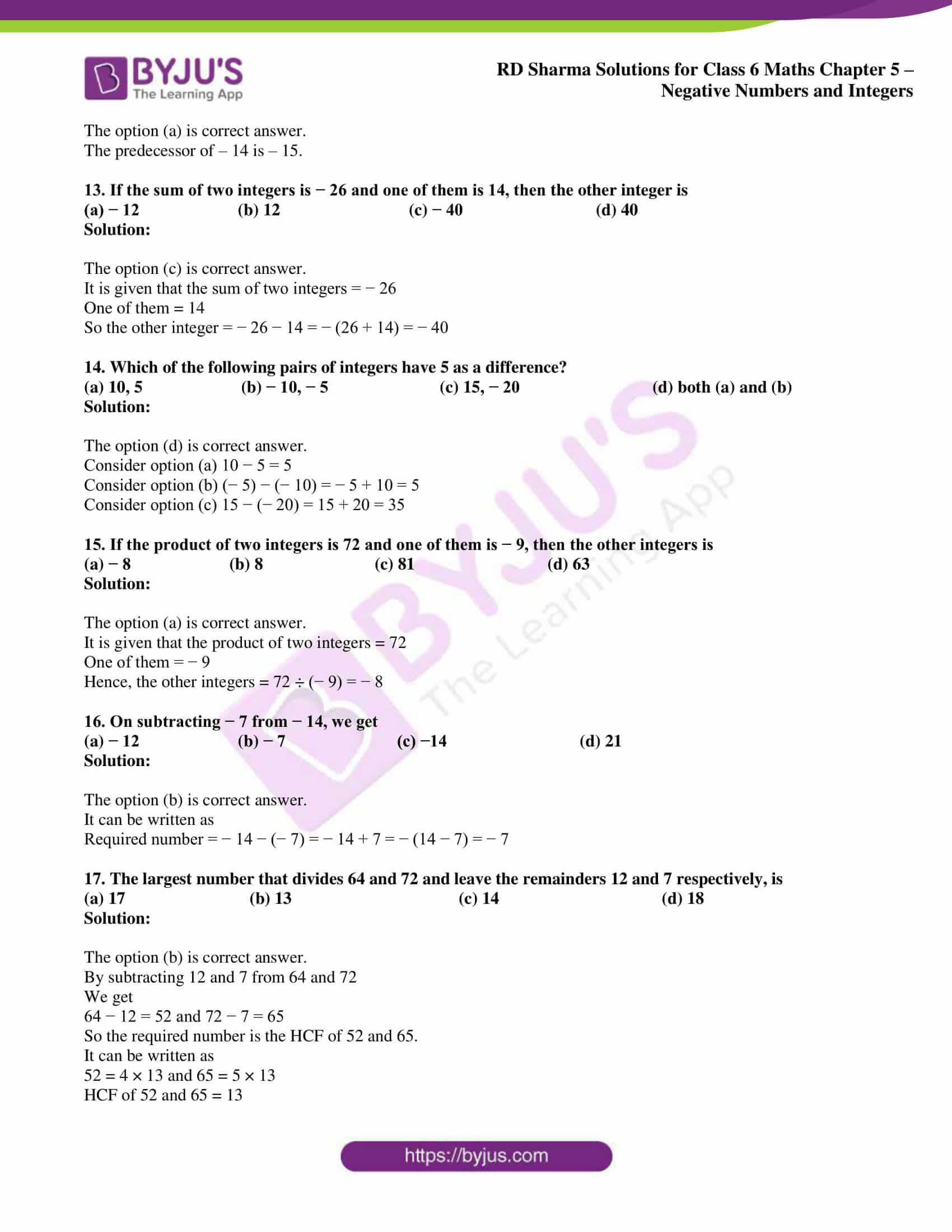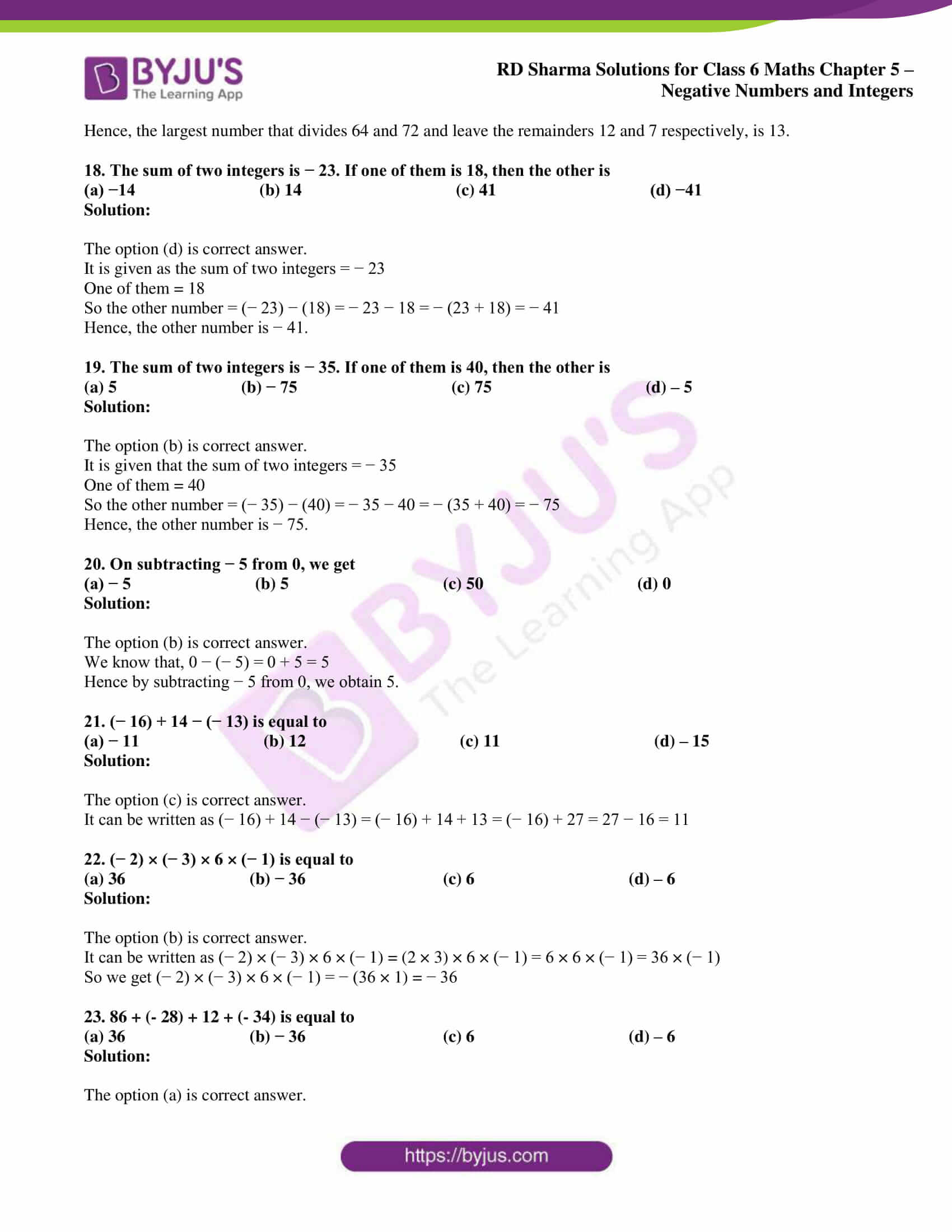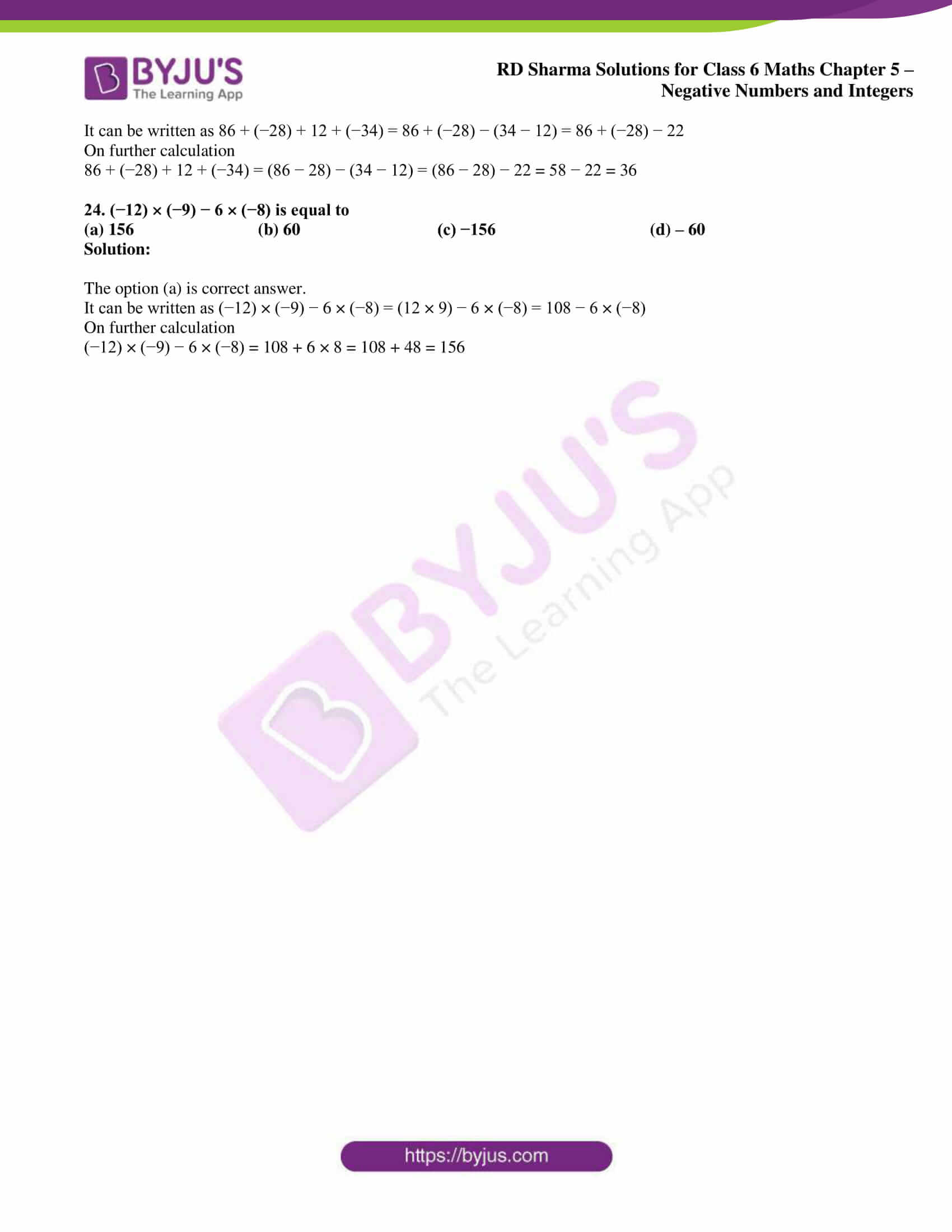## Access answers to Maths RD Sharma Solutions for Class 6 Chapter 5: Negative Numbers and Integers

### Exercise 5.1 PAGE: 5.5

1. Write the opposite of each of the following:

(i) Increase in population

(ii) Depositing money in a bank

(iii) Earning money

(iv) Going North

(v) Gaining a weight of 4kg

(vi) A loss of Rs 1000

(vii) 25

(viii) – 15

Solution:

(i) The opposite of Increase in population is Decrease in population.

(ii) The opposite of Depositing money in a bank is Withdrawing money from a bank.

(iii) The opposite of earning money is Spending money.

(iv) The opposite of Going North is Going South.

(v) The opposite of gaining a weight of 4kg is losing a weight of 4kg.

(vi) The opposite of a loss of Rs 1000 is a gain of Rs 1000.

(vii) The opposite of 25 is – 25.

(viii) The opposite of – 15 is 15.

2. Indicate the following by using integers:

(i) 25o above zero

(ii) 5o below zero

(iii) A profit of Rs 800

(iv) A deposit of Rs 2500

(v) 3km above sea level

(vi) 2km below level

Solution:

(i) 25o above zero is + 25o.

(ii) 5o below zero is – 5o.

(iii) A profit of Rs 800 is + 800.

(iv) A deposit of Rs 2500 is + 2500.

(v) 3km above sea level is + 3.

(vi) 2km below level is – 2.

3. Mark the following integers on a number line:

(i) 7

(ii) -4

(iii) 0

Solution:

The following integers are marked on a number line as given below: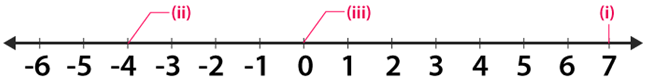4. Which number in each of the following pairs is smaller?

(i) 0, -4

(ii) -3 , 12

(iii) 8, 13

(iv) – 15, -27

Solution:

(i) 0 is greater than the negative integers

So we get – 4 < 0

Therefore, – 4 is smaller.

(ii) 12 is greater than -3 on a number line

So we get

-3 < 12

Therefore, – 3 is smaller.

(iii) 13 is greater than 8 on a number line

So we get 8 < 13

Therefore, 8 is smaller.

(iv) – 15 is greater than – 27 on a number line

So we get – 27 < – 15

Therefore, – 27 is smaller.

5. Which number in each of the following pairs is larger?

(i) 3, -4

(ii) – 12, – 8

(iii) 0, 7

(iv) 12, – 18

Solution:

(i) We know that 3 is larger than – 4 on a number line

So we get 3 > – 4

Therefore, 3 is larger.

(ii) We know that – 8 is larger than – 12 on a number line

So we get – 8 > – 12

Therefore, – 8 is larger.

(iii) We know that 7 is larger than 0 on a number line

So we get 7 > 0

Therefore, 7 is larger.

(iv) We know that 12 is larger than – 18 on a number line

So we get 12 > – 18

Therefore, 12 is larger.

6. Write all integers between:

(i) – 7 and 3

(ii) – 2 and 2

(iii) – 4 and 0

(iv) 0 and 3

Solution:

(i) The integers between – 7 and 3 are

– 6, – 5, – 4, – 3, – 2, – 1, 0, 1, 2

(ii) The integers between – 2 and 2 are

-1, 0, 1.

(iii) The integers between – 4 and 0 are

-3, -2, -1

(iv) The integers between 0 and 3 are

1, 2.

7. How many integers are between?

(i) – 4 and 3

(ii) 5 and 12

(iii) – 9 and – 2

(iv) 0 and 5

Solution:

(i) The integers between – 4 and 3 are

-3, -2, -1, 0, 1, 2

Therefore, number of integers between – 4 and 3 are 6.

(ii) The integers between 5 and 12 are

6, 7, 8, 9, 10, 11

Therefore, number of integers between 5 and 12 are 6.

(iii) The integers between – 9 and – 2 are

-8, -7, -6, -5, -4, -3

Therefore, number of integers between -9 and -2 are 6.

(iv) The integers between 0 and 5 are

1, 2, 3, 4

Therefore, number of integers between 0 and 5 are 4.

8. Replace * in each of the following by < or > so that the statement is true:

(i) 2 * 5

(ii) 0 * 3

(iii) 0 * – 7

(iv) – 18 * 15

(v) – 235 * – 532

(vi) – 20 * 20

Solution:

(i) 2 < 5

(ii) 0 < 3

(iii) 0 > – 7

(iv) – 18 < 15

(v) – 235 > – 532

(vi) – 20 < 20

9. Write the following integers in increasing order:

(i) – 8, 5, 0, -12, 1, -9, 15

(ii) – 106, 107, – 320, – 7, 185

Solution:

(i) – 8, 5, 0, -12, 1, -9, 15 can be written in increasing order as

– 12, – 9, – 8, 0, 1, 5, 15

(ii) – 106, 107, – 320, – 7, 185 can be written in increasing order as

-320, – 106, – 7, 107, 185.

10. Write the following integers in decreasing order:

(i) – 15, 0, -2, -9, 7, 6, -5, 8

(ii) -154, 123, -205, -89, -74

Solution:

(i) – 15, 0, -2, -9, 7, 6, -5, 8 can be written in decreasing order as

8, 7, 6, 0, -2, -5, -9, -15

(ii) -154, 123, -205, -89, -74 can be written in decreasing order as

123, – 74, – 89, – 154, – 205

11. Using the number line, write the integer which is:

(i) 2 more than 3

(ii) 5 less than 3

(iii) 4 more than – 9

Solution:

(i) 2 more than 3

In order to get the integer 2 more than 3

We draw a number line from 2 and proceed 3 units to the right to obtain 5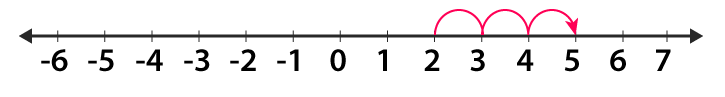Therefore, 2 more than 3 is 5.

(ii) 5 less than 3

In order to get the integer 5 less than 3

We draw a number line from 3 and proceed 5 units to the left to obtain – 2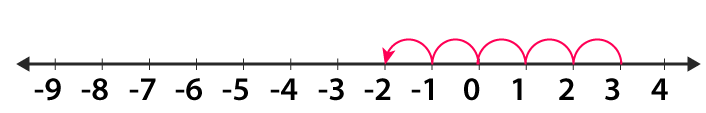Therefore, 5 less than 3 is – 2.

(iii) 4 more than – 9

In order to get the integer 4 more than – 9

We draw a number line from – 9 and proceed 4 units to the right to obtain -5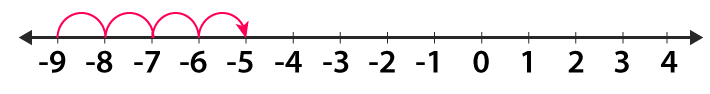Therefore, 4 more than – 9 is – 5.

12. Write the absolute value of each of the following:

(i) 14

(ii) – 25

(iii) 0

(iv) – 125

(v) – 248

(vi) a – 7, if a is greater than 7

(vii) a – 7, if a – 2 is less than 7

(viii) a + 4, if a is greater than -4

(ix) a + 4 if a is less than – 4

(x) |-3|

(xi) -|-5|

(xii) |12 – 5|

Solution:

(i) The absolute value of 14 is

|14| = 14

(ii) The absolute value of – 25 is

|-25| = 25

(iii) The absolute value of 0 is

|0| = 0

(iv) The absolute value of – 125 is

|-125| = 125

(v) The absolute value of – 248 is

|-248| = 248

(vi) The absolute value of a – 7, if a is greater than 7 is

|a – 7| = a – 7 where a > 7

(vii) The absolute value of a – 7, if a – 2 is less than 7 is

|a – 7| = – (a – 7) where a – 2 < 7

(viii) The absolute value of a + 4, if a is greater than -4 is

|a + 4| = a + 4 where a > – 4

(ix) The absolute value of a + 4 if a is less than – 4 is

|a + 4| = – (a + 4) where a < -4

(x) The absolute value of |-3| is

|-3| = 3

(xi) The absolute value of -|-5| is

-|-5| = 5

(xii) The absolute value of |12 – 5| is

|12 – 5| = 7

13. (i) Write 4 negative integers less than – 10.

(ii) Write 6 negative integers just greater than – 12.

Solution:

(i) The 4 negative integers less than – 10 are

– 11, – 12, – 13, – 14

(ii) The 6 negative integers just greater than – 12 are

-11, – 10, – 9, – 8, – 7, – 6

14. Which of the following statements are true?

(i) The smallest integer is zero.

(ii) The opposite of zero is zero.

(iii) Zero is not an integer.

(iv) 0 is larger than every negative integer.

(v) The absolute value of an integer is greater than the integer.

(vi) A positive integer is greater than its opposite.

(vii) Every negative integer is less than every natural number.

(viii) 0 is the smallest positive integer.

Solution:

(i) False. The smallest integer is 1.

(ii) True. 0 is neither positive nor negative so the opposite is 0.

(iii) False. Zero is an integer which is neither positive nor negative.

(iv) True. 0 is larger than – 1.

(v) False. The absolute value of an integer is the numerical value.

(vi) True. 3 is greater than – 3.

(vii) True. – 3 is less than 1.

(viii) False. 1 is the smallest positive integer.

### Exercise 5.2 PAGE: 5.9

1. Draw a number line and represent each of the following on it:

(i) 5 + (-2)

(ii) (-9) + 4

(iii) (-3) + (-5)

(iv) 6 + (-6)

(v) (-1) + (-2) + 2

(vi) (-2) + 7 + (-9)

Solution:

(i) 5 + (-2)

From 0 move towards right of first five units to obtain + 5

So the second number is – 2 so move 2 units towards left of + 5 we get + 3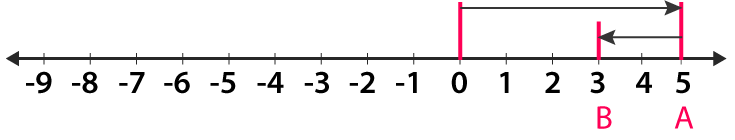Therefore, 5 + (-2) = 3.

(ii) (-9) + 4

From 0 move towards left of nine units to obtain – 9

So the second number is 4 so move 4 units towards right of – 9 we get – 5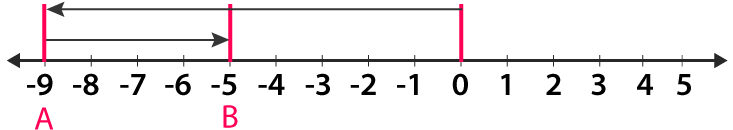Therefore, (-9) + 4 = – 5.

(iii) (-3) + (-5)

From 0 move towards left of three units to obtain – 3

So the second number is – 5 so move 5 units towards left of – 3 we get – 8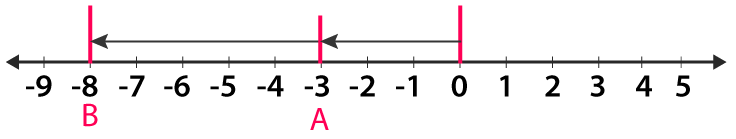Therefore, (-3) + (-5) = – 8.

(iv) 6 + (-6)

From zero move towards right of six units to obtain 6

So the second number is – 6 so move 6 units towards left of 6 we get 0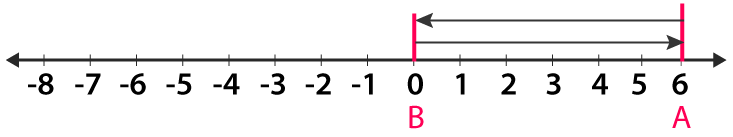Therefore, 6 + (-6) = 0.

(v) (-1) + (-2) + 2

From zero move towards left of one unit to obtain – 1

So the second number is – 2 so move 2 units towards left of – 1 we get – 3

The third number is 2 so move 2 units towards right of – 3 we get – 1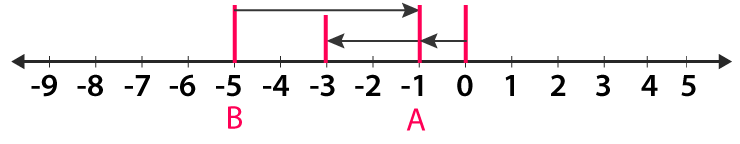Therefore, (-1) + (-2) + 2 = – 1.

(vi) (-2) + 7 + (-9)

From zero move towards left of two units to obtain – 2

So the second number is 7 so move 7 units towards right of – 2 we get 5

The third number is – 9 so move 9 units towards left of 5 we get – 4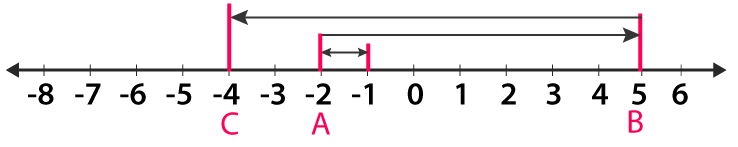Therefore, (-2) + 7 + (-9) = – 4.

2. Find the sum of

(i) -557 and 488

(ii) -522 and -160

(iii) 2567 and – 325

(iv) -10025 and 139

(v) 2547 and -2548

(vi) 2884 and -2884

Solution:

(i) -557 and 488

We get

-557 + 488

It can be written as

|-557| – |488| = 557 – 488 = 69.

(ii) -522 and -160

We get

-522 + (-160)

It can be written as

-522 – 160 = – 682

(iii) 2567 and – 325

We get

2567 + (-325)

It can be written as

2567 – 325 = 2242

(iv) -10025 and 139

We get

-10025 + 139

It can be written as

-10025 + 139 = -9886

(v) 2547 and -2548

We get

2547 + (-2548)

It can be written as

2547 – 2548 = -1

(vi) 2884 and -2884

We get

2884 + (-2884)

It can be written as

2884 – 2884 = 0

### Exercise 5.3 page: 5.11

1. Find the additive inverse of each of the following integers:

(i) 52

(ii) – 176

(iii) 0

(iv) 1

Solution:

(i) The additive inverse of 52 is – 52.

(ii) The additive inverse of – 176 is 176.

(iii) The additive inverse of 0 is 0.

(iv) The additive inverse of 1 is – 1.

2. Find the successor of each of the following integers:

(i) – 42

(ii) -1

(iii) 0

(iv) – 200

(v) -99

Solution:

(i) The successor of – 42 is

– 42 + 1 = – 41

(ii) The successor of – 1 is

-1 + 1 = 0

(iii) The successor of 0 is

0 + 1 = 1

(iv) The successor of – 200 is

-200 + 1 = – 199

(v) The successor of – 99 is

– 99 + 1 = – 98

3. Find the predecessor of each of the following integers:

(i) 0

(ii) 1

(iii) – 1

(iv) – 125

(v) 1000

Solution:

(i) The predecessor of 0 is

0 – 1 = – 1

(ii) The predecessor of 1 is

1 – 1 = 0

(iii) The predecessor of -1 is

-1 – 1 = -2

(iv) The predecessor of – 125 is

-125 – 1 = – 126

(v) The predecessor of 1000 is

1000 – 1 = 999

4. Which of the following statements are true?

(i) The sum of a number and its opposite is zero.

(ii) The sum of two negative integers is a positive integer.

(iii) The sum of a negative integer and a positive integer is always a negative integer.

(iv) The successor of – 1 is 1.

(v) The sum of three different integers can never be zero.

Solution:

(i) True. 1 – 1 = 0

(ii) False. -1 – 1 = -2

(iii) False. – 2 + 3 = 1

(iv) False. The successor of – 1 is 0.

(v) False. 1 + 2 – 3 = 0

5. Write all integers whose absolute values are less than 5.

Solution:

The integers whose absolute values are less than 5 are

-4, – 3, – 2, – 1, 0, 1, 2, 3, 4

6. Which of the following is false:

(i) |4 + 2| = |4| + |2|

(ii) |2 – 4| = |2| + |4|

(iii) |4 – 2| = |4| – |2|

(iv) |(-2) + (-4)| = |-2| + |-4|

Solution:

(i) True.

(ii) False.

(iii) True.

(iv) True.

7. Complete the following table: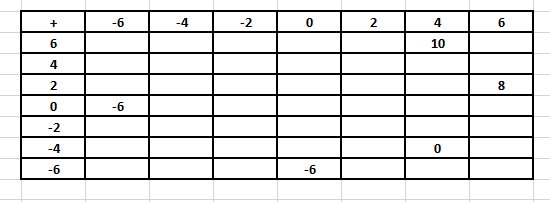From the above table:

(i) Write all the pairs of integers whose sum is 0.

(ii) Is (-4) + (-2) = (-2) + (-4)?

(iii) Is 0 + (-6) = -6?

Solution: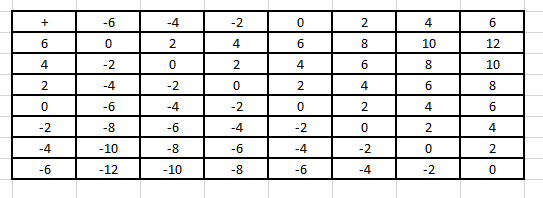(i) The pairs of integers whose sum is 0 are

(6, -6), (4, – 4), (2, – 2), (0, 0)

(ii) Yes. By using commutativity of addition (-4) + (-2) = (-2) + (-4)

(iii) Yes. By using additive identity 0 + (-6) = -6.

8. Find an integer x such that

(i) x + 1 = 0

(ii) x + 5 = 0

(iii) – 3 + x = 0

(iv) x + (-8) = 0

(v) 7 + x = 0

(vi) x + 0 = 0

Solution:

(i) x + 1 = 0

Subtracting 1 on both sides

x + 1 – 1 = 0 – 1

We get

x = -1

(ii) x + 5 = 0

By subtracting 5 on both sides

x + 5 – 5 = 0 – 5

So we get

x = -5

(iii) – 3 + x = 0

By adding 3 on both sides

-3 + x + 3 = 0 + 3

So we get

x = 3

(iv) x + (-8) = 0

By adding 8 on both sides

x – 8 + 8 = 0 + 8

So we get

x = 8

(v) 7 + x = 0

By subtracting 7 on both sides

7 + x – 7 = 0 – 7

So we get

x = – 7

(vi) x + 0 = 0

So we get

x = 0

### Exercise 5.4 page: 5.17

1. Subtract the first integer from the second in each of the following:

(i) 12, -5

(ii) – 12, 8

(iii) – 225, – 135

(iv) 1001, 101

(v) – 812, 3126

(vi) 7560, – 8

(vii) – 3978, – 4109

(viii) 0, – 1005

Solution:

(i) 12, -5

So by subtracting the first integer from the second

-5 – 12 = – 17

(ii) – 12, 8

So by subtracting the first integer from the second

8 – (-12) = 8 + 12 = 20

(iii) – 225, – 135

So by subtracting the first integer from the second

-135 – (-225) = 225 – 135 = 90

(iv) 1001, 101

So by subtracting the first integer from the second

101 – 1001 = – 900

(v) – 812, 3126

So by subtracting the first integer from the second

3126 – (-812) = 3126 + 812 = 3938

(vi) 7560, – 8

So by subtracting the first integer from the second

-8 – 7560 = – 7568

(vii) – 3978, – 4109

So by subtracting the first integer from the second

-4109 – (-3978) = – 4109 + 3978 = -131

(viii) 0, – 1005

So by subtracting the first integer from the second

-1005 – 0 = – 1005

2. Find the value of:

(i) – 27 – (- 23)

(ii) – 17 – 18 – (-35)

(iii) – 12 – (-5) – (-125) + 270

(iv) 373 + (-245) + (-373) + 145 + 3000

(v) 1 + (-475) + (-475) + (-475) + (-475) + 1900

(vi) (-1) + (-304) + 304 + 304 + (-304) + 1

Solution:

(i) – 27 – (- 23)

So we get

= – 27 + 23

On further calculation

= 23 – 27

We get

= – 4

(ii) – 17 – 18 – (-35)

So we get

= – 35 + 35

On further calculation

= 0

(iii) – 12 – (-5) – (-125) + 270

So we get

= – 12 + 5 + 125 + 270

On further calculation

= 400 – 12

We get

= 388

(iv) 373 + (-245) + (-373) + 145 + 3000

So we get

= 373 – 245 – 373 + 145 + 3000

On further calculation

= 3145 + 373 – 373 – 245

We get

= 3145 – 245

By subtraction

= 2900

(v) 1 + (-475) + (-475) + (-475) + (-475) + 1900

So we get

= 1 – 950 – 950 + 1900

On further calculation

= 1900 + 1 – 1900

We get

= 1

(vi) (-1) + (-304) + 304 + 304 + (-304) + 1

So we get

= – 1 + 1 – 304 + 304 – 304 + 304

On further calculation

= 0

3. Subtract the sum of – 5020 and 2320 from – 709.

Solution:

We know that the sum of -5020 and 2320 is

-5020 + 2320

It can be written as

= 2320 – 5020

So we get

= – 2700

Subtracting from – 709 we get

= – 709 – (-2700)

We get

= – 709 + 2700

By subtraction

= 1991

4. Subtract the sum of – 1250 and 1138 from the sum of 1136 and – 1272.

Solution:

We know that the sum of – 1250 and 1138 is

-1250 + 1138

It can be written as

= 1138 – 1250

So we get

= – 112

We know that the sum of 1136 and – 1272 is

1136 – 1272 = – 136

So we get

-136 – (-112) = – 136 + 112 = -24

5. From the sum of 233 and – 147, subtract – 284.

Solution:

We know that the sum of 233 and – 147 is

233 – 147 = 86

Subtracting – 284 we get

86 – (-284) = 86 + 284 = 370

6. The sum of two integers is 238. If one of the integers is – 122, determine the other.

Solution:

It is given that

Sum of two integers = 238

One of the integers = – 122

So the other integer = – (-122) + 238

On further calculation

Other integer = 238 + 122 = 360

7. The sum of two integers is – 223. If one of the integers is 172, find the other.

Solution:

It is given that

Sum of two integers = – 223

One of the integers = 172

So the other integer = – 223 – 172 = – 395

8. Evaluate the following:

(i) – 8 – 24 + 31 – 26 – 28 + 7 + 19 – 18 – 8 + 33

(ii) – 26 – 20 + 33 – (-33) + 21 + 24 – (-25) – 26 – 14 – 34

Solution:

(i) – 8 – 24 + 31 – 26 – 28 + 7 + 19 – 18 – 8 + 33

We get

= – 8 – 24 – 26 – 28 – 18 – 8 + 31 + 7 + 19 + 33

On further calculation

= – 32 – 26 – 28 – 26 + 38 + 19 + 33

It can be written as

= 38 – 32 – 26 – 28 + 33 – 26 + 19

So we get

= 6 – 26 – 28 + 7 + 19

By calculation

= 6 – 28 – 26 + 26

= 6 – 28

By subtraction

= – 22

(ii) – 26 – 20 + 33 – (-33) + 21 + 24 – (-25) – 26 – 14 – 34

We get

= – 46 + 33 + 33 + 21 + 24 + 25 – 26 – 14 – 34

On further calculation

= – 46 + 66 + 21 + 24 + 25 + (-74)

It can be written as

= – 46 + 66 + 70 – 74

So we get

= – 46 – 4 + 66

By calculation

= – 50 + 66

= 66 – 50

By subtraction

= 16

9. Calculate

1 – 2 + 3 – 4 + 5 – 6 + ……… + 15 – 16

Solution:

It can be written as

1 – 2 + 3 – 4 + 5 – 6 + 7 – 8 + 9 – 10 + 11 – 12 + 13 – 14 + 15 – 16

We get

= – 1 – 1 – 1 – 1 – 1 – 1 – 1 – 1

By calculation

= – 8

10. Calculate the sum:

5 + (-5) + 5 + (-5) + …..

(i) if the number of terms is 10.

(ii) if the number of terms is 11.

Solution:

(i) if the number of terms is 10

We get

5 + (-5) + 5 + (-5) + 5 + (-5) + 5 + (-5) + 5 + (-5)

On further calculation

= 5 – 5 + 5 – 5 + 5 – 5 + 5 – 5 + 5 – 5 = 0

(ii) if the number of terms is 11

We get

5 + (-5) + 5 + (-5) + 5 + (-5) + 5 + (-5) + 5 + (-5) + 5

On further calculation

= 5 – 5 + 5 – 5 + 5 – 5 + 5 – 5 + 5 – 5 + 5 = 5

11. Replace * by < or > in each of the following to make the statement true:

(i) (-6) + (-9) * (-6) – (-9)

(ii) (-12) – (-12) * (-12) + (-12)

(iii) (-20) – (-20) * 20 – (65)

(iv) 28 – (-10) * (-16) – (-76)

Solution:

(i) (-6) + (-9) < (-6) – (-9)

(ii) (-12) – (-12) > (-12) + (-12)

(iii) (-20) – (-20) > 20 – (65)

(iv) 28 – (-10) < (-16) – (-76)

12. If △ is an operation on integers such that a △ b = – a + b – (-2) for all integers a, b. Find the value of

(i) 4 △ 3

(ii) (-2) △ (-3)

(iii) 6 △ (-5)

(iv) (-5) △ 6

Solution:

(i) 4 △ 3

By substituting values in a △ b = – a + b – (-2)

We get

4 △ 3 = – 4 + 3 – (-2) = 1

(ii) (-2) △ (-3)

By substituting values in a △ b = – a + b – (-2)

We get

(-2) △ (-3) = – (-2) + (-3) – (-2) = 1

(iii) 6 △ (-5)

By substituting values in a △ b = – a + b – (-2)

We get

6 △ (-5) = – 6 + (-5) – (-2) = – 9

(iv) (-5) △ 6

By substituting values in a △ b = – a + b – (-2)

We get

(-5) △ 6 = – (-5) + 6 – (-2) = 13

13. If a and b are two integers such that a is the predecessor of b. Find the value of a – b.

Solution:

It is given that a is the predecessor of b

We can write it as

a + 1 = b

So we get

a – b = – 1

14. If a and b are two integers such that a is the successor of b. Find the value of a – b.

Solution:

It is given that a is the successor of b

We can write it as

a – 1 = b

So we get

a – b = 1

15. Which of the following statements are true:

(i) – 13 > – 8 – (-2)

(ii) – 4 + (-2) < 2

(iii) The negative of a negative integer is positive.

(iv) If a and b are two integers such that a > b, then a – b is always a positive integer.

(v) The difference of two integers is an integer.

(vi) Additive inverse of a negative integer is negative.

(vii) Additive inverse of a positive integer is negative.

(viii) Additive inverse of a negative integer is positive.

Solution:

(i) False.

(ii) True.

(iii) True.

(iv) True.

(v) True.

(vi) False.

(vii) True.

(viii) True.

16. Fill in the blanks:

(i) – 7 + ….. = 0

(ii) 29 + ….. = 0

(iii) 132 + (-132) = ….

(iv) – 14 + ….. = 22

(v) – 1256 + ….. = – 742

(vi) ….. – 1234 = – 4539

Solution:

(i) – 7 + 7 = 0

(ii) 29 + (-29) = 0

(iii) 132 + (-132) = 0

(iv) – 14 + 36 = 22

(v) – 1256 + 514 = – 742

(vi) -3305 – 1234 = – 4539

### Objective Type Questions page: 5.18

Mark the correct alternative in each of the following:

1. Which of the following statement is true?
(a) − 7 > − 5              (b) − 7 < − 5                 (c) (− 7) + (− 5) > 0                       (d) (− 7) − (− 5) > 0

Solution:

The option (b) is correct answer.

In option (a)

We know that − 7 is to the left of – 5

Hence, − 7 < − 5.

In option (c)

We know that (− 7) + (− 5) = − (7 + 5) = − 12.

So − 12 is to the left of 0

Hence (− 7) + (− 5) < 0.

In option (d)

(− 7) − (− 5) = (− 7) + (additive inverse of − 5) = (− 7) + (5) = − (7 − 5) = − 2

We know that − 2 is to the left of 0, so (− 7) − (− 5) < 0.

2. 5 less than − 2 is
(a) 3                            (b) − 3                        (c) − 7                                 (d) 7

Solution:

The option (c) is correct answer.

We know that, 5 less than − 2 = (− 2) − (5) = − 2 − 5 = − 7

3. 6 more than − 7 is
(a) 1                            (b) − 1                        (c) 13                                 (d) – 13

Solution:

The option (b) is correct answer.

We know that, 6 more than − 7 = (− 7) + 6 = − (7 − 6) = − 1

4. If x is a positive integer, then
(a) x + |x| = 0              (b) x − |x| = 0                    (c) x + |x| = −2x                (d) x = − |x|

Solution:

The option (b) is correct answer.

We know that if x is positive integer, then |x| = x

Hence, x + |x| = x + x = 2x and x − |x| = x − x = 0

5. If x is a negative integer, then
(a) x + |x| = 0              (b) x − |x| = 0                    (c) x + |x| = 2x                (d) x − |x| = − 2x

Solution:

The option (a) is correct answer.

We know that x is negative integer, then |x| = −x

It can be written as

x + |x| = x − x = 0 and x − |x| = x − (− x) = x + x = 2x

6. If x is greater than 2, then |2 − x| =
(a) 2 − x              (b) x − 2                    (c) 2 + x                (d) − x – 2

Solution:

The option (b) is correct answer.

We know that if a is negative integer, then |a| = − a

It is given that x is greater than 2 where 2 − x is negative

Hence, |2 − x| = − (2 − x) = − 2 + x = x − 2.

7. 9 + |− 4| is equal to
(a) 5                         (b) − 5                       (c) 13                            (d) −13

Solution:

The option (c) is correct answer.

We know that, |− 4| = 4

Hence 9 + |− 4| = 9 + 4 = 13

8. (− 35) + (− 32) is equal to
(a) 67                         (b) − 67                       (c) − 3                            (d) 3

Solution:

The option (b) is correct answer.

It can be written as (− 35) + (− 32) = − (35 + 32) = − 67

9. (− 29) + 5 is equal to
(a) 24                         (b) 34                       (c) − 34                            (d) – 24

Solution:

The option (d) is correct answer.

It can be written as (− 29) + 5 = − (29 − 5) = − 24

10. |− |− 7| − 3| is equal to
(a) − 7                       (b) 7                              (c) 10                                    (d) – 10

Solution:

The option (c) is correct answer.

It can be written as |− |− 7| − 3| = |− 7 − 3| = |− 10| = 10

11. The successor of − 22 is
(a) − 23                       (b) − 21                              (c) 23                                    (d) 21

Solution:

The option (b) is correct answer.

We know that if ‘a’ is an integer a + 1 is its successor.

So the successor of − 22 = − 22 + 1 = − (22 − 1) = − 21

12. The predecessor of – 14 is

(a) – 15 (b) 15 (c) 13 (d) – 13

Solution:

The option (a) is correct answer.

The predecessor of – 14 is – 15.

13. If the sum of two integers is − 26 and one of them is 14, then the other integer is
(a) − 12                       (b) 12                              (c) − 40                               (d) 40

Solution:

The option (c) is correct answer.

It is given that the sum of two integers = − 26

One of them = 14

So the other integer = − 26 − 14 = − (26 + 14) = − 40

14. Which of the following pairs of integers have 5 as a difference?
(a) 10, 5                       (b) − 10, − 5                          (c) 15, − 20                               (d) both (a) and (b)

Solution:

The option (d) is correct answer.

Consider option (a) 10 − 5 = 5

Consider option (b) (− 5) − (− 10) = − 5 + 10 = 5

Consider option (c) 15 − (− 20) = 15 + 20 = 35

15. If the product of two integers is 72 and one of them is − 9, then the other integers is
(a) − 8                       (b) 8                          (c) 81                               (d) 63

Solution:

The option (a) is correct answer.

It is given that the product of two integers = 72

One of them = − 9

Hence, the other integers = 72 ÷ (− 9) = − 8

16. On subtracting − 7 from − 14, we get
(a) − 12                       (b) − 7                          (c) −14                               (d) 21

Solution:

The option (b) is correct answer.

It can be written as

Required number = − 14 − (− 7) = − 14 + 7 = − (14 − 7) = − 7

17. The largest number that divides 64 and 72 and leave the remainders 12 and 7 respectively, is
(a) 17                             (b) 13                                       (c) 14                                      (d) 18

Solution:

The option (b) is correct answer.

By subtracting 12 and 7 from 64 and 72

We get

64 − 12 = 52 and 72 − 7 = 65

So the required number is the HCF of 52 and 65.

It can be written as

52 = 4 × 13 and 65 = 5 × 13

HCF of 52 and 65 = 13

Hence, the largest number that divides 64 and 72 and leave the remainders 12 and 7 respectively, is 13.

18. The sum of two integers is − 23. If one of them is 18, then the other is
(a) −14                             (b) 14                                    (c) 41                                    (d) −41

Solution:

The option (d) is correct answer.

It is given as the sum of two integers = − 23

One of them = 18

So the other number = (− 23) − (18) = − 23 − 18 = − (23 + 18) = − 41

Hence, the other number is − 41.

19. The sum of two integers is − 35. If one of them is 40, then the other is
(a) 5                             (b) − 75                                    (c) 75                                    (d) – 5

Solution:

The option (b) is correct answer.

It is given that the sum of two integers = − 35

One of them = 40

So the other number = (− 35) − (40) = − 35 − 40 = − (35 + 40) = − 75

Hence, the other number is − 75.

20. On subtracting − 5 from 0, we get
(a) − 5                             (b) 5                                    (c) 50                                    (d) 0

Solution:

The option (b) is correct answer.

We know that, 0 − (− 5) = 0 + 5 = 5

Hence by subtracting − 5 from 0, we obtain 5.

21. (− 16) + 14 − (− 13) is equal to
(a) − 11                             (b) 12                                    (c) 11                                    (d) – 15

Solution:

The option (c) is correct answer.

It can be written as (− 16) + 14 − (− 13) = (− 16) + 14 + 13 = (− 16) + 27 = 27 − 16 = 11

22. (− 2) × (− 3) × 6 × (− 1) is equal to
(a) 36                             (b) − 36                                (c) 6                                    (d) – 6

Solution:

The option (b) is correct answer.

It can be written as (− 2) × (− 3) × 6 × (− 1) = (2 × 3) × 6 × (− 1) = 6 × 6 × (− 1) = 36 × (− 1)

So we get (− 2) × (− 3) × 6 × (− 1) = − (36 × 1) = − 36

23. 86 + (- 28) + 12 + (- 34) is equal to
(a) 36                             (b) − 36                                (c) 6                                    (d) – 6

Solution:

The option (a) is correct answer.

It can be written as 86 + (−28) + 12 + (−34) = 86 + (−28) − (34 − 12) = 86 + (−28) − 22

On further calculation

86 + (−28) + 12 + (−34) = (86 − 28) − (34 − 12) = (86 − 28) − 22 = 58 − 22 = 36

24. (−12) × (−9) − 6 × (−8) is equal to
(a) 156                             (b) 60                                (c) −156                                    (d) – 60

Solution:

The option (a) is correct answer.

It can be written as (−12) × (−9) − 6 × (−8) = (12 × 9) − 6 × (−8) = 108 − 6 × (−8)

On further calculation

(−12) × (−9) − 6 × (−8) = 108 + 6 × 8 = 108 + 48 = 156

### RD Sharma Solutions for Class 6 Maths Chapter 5: Negative Numbers and Integers

Chapter 5, Negative Numbers and Integers, has 4 exercises with problems based on types of integers and operations. RD Sharma Solutions Chapter 5 contains some of the important concepts which are listed below:

• The Need for Integers
• Introduction of Integers
• Representation of Integers on Number Line
• Ordering of Integers
• Negative of a Negative Integer
• Absolute Value of an Integer